UNIVERSITÀ DEGLI STUDI DI BOLOGNA

### Abstract

This dissertation addresses interference problem in Global Navigation Satellite System (GNSS) receivers as dominant topic. The term interference opens numerous list of topics which shows growing concerns that this problem caused in the recent years. The European GNSS agency (GSA) estimated in their fourth comprehensive report that there were more than 3.6 billion GNSS devices in use around the globe in 2014 and expected to double by 2019. Smartphones, tablets, tracking devices, digital cameras, portable computers, fitness gear, and more all use GNSS technology for navigation, mapping and determining consumer preferences. More so, safety applications benefit from accurate emergency caller location tracking and location based services (LBS), which provides services to enterprisers and ordinary users. Consequently, investigating sources of any disturbances on GNSS devices is very important. Secure and reliable GNSS solutions is the core demand of market these days. Although many traditional solutions proposed in GNSS literature, but still many open issues exist in this area. This Thesis pursues a twofold objective: on the one hand, it introduce detection, mitigation and localization techniques in GNSS in theoretical perspective, and on the other hand it propose an experiment in real environment using those information plus a novel joint jammer detection and localization algorithm. In this dissertation we briefly describe GNSS concepts, signals and systems and then in detail, we explain interference classification where various type of interference taken into account. Our concentration in this dissertation is on jamming type of interference. Surveying some jamming incidents along with scientific facts can prove the high necessity of interference confrontation. After explaining the basic concepts, we will introduce detection techniques, since detecting interference is not enough to avoid disturbances on receiver and stabilize performance therefore, we will initiate mitigation techniques to explain countering methods to interference threats and refine interfered and disturbed signal.

Next part of the dissertation, dedicated to a novel algorithm for jointly detecting and localizing interference source, where a network of sensing nodes collecting data from an area and feeding them to a central unit equipped with an algorithm that take advantage of an Extended Kalman Filter (EKF) and takes an aggregate decision on jamming existence, after decision had been made, the localization starts with help of another EKF, which led us to introduce Kalamn filter theory. Algorithm description and results rooted from theoretical implementation of algorithm consist the last part of the referred chapter. Proving the whole theory behind this novel algorithm in real environment with software defined radio equipment, is the purpose of the last chapter, recording results, comparing them with each other and finally give receiver operating characteristic as an indicator of optimal Kalman filter configuration are the sections consisting the last chapter. Correctly optimizing parameters of Kalman filter in different scenario and environments depicted in the last chapter. At the end, conclusion on the whole dissertation and suggestions for future works expressed.

### Acknowledgements

I would like to thank my advisor, Professor Giovanni E. Corazza, for providing me the opportunity to pursue this research topic. His technical insight and leading skills have been a great source of inspiration to me. Additionally, his skills as a teacher, advisor and mentor during two years of my master degree are much appreciated. Also I would like to thank Dr. Marco Bartolucci and Dr. Giacomo Pojani for always making time to provide me with their guidance, technical expertise and comments on my thesis that helped me to overcome difficulties in the past six months. I would also like to thank Professor Gianni Pasolini who introduced me to new era of communication systems equipment which I used in my experiment of this dissertation his warm support also appreciated.

The Department of Electronic and Information Engineering (DEI) and University of Bologna are gratefully acknowledged for establishing the best possible environment for the pursuit of graduate studies.

Last but not least, I would like to express my highest respect and appreciation to my parents and brother who support me during all these years mentally and spiritually.

Abstract iii

Acknowledgments iv

List of figures and illustrations viii

List of tables ix

Chapter 1:

1. Global Navigation Satellite System (GNSS) 1

1. Overview and concept 1

1. GNSS instances 2

2. Global Positioning System (GPS) 3

1. Signal characteristic 3

3. GNSS receiver, antennas and frontends 5

1. Acquisition and tracking 6

3. Pseudorange and user position computation 9

Chapter 2:

2. Interference in GNSS 12

1. Interference classification 12

1. Wideband and narrowband interference 12

2. Jamming interference 14

3. Spoofing interference 14

4. Meaconing interference 15

2. Example of jamming incidents 15

3. Interference detection 16

1. Basics of detection theory 17

2. Energy detection 19

3. C/N0 based detection 21

4. Detection based on antenna array 22

5. Detection based on GNSS/INS integration 24

6. Other detection methods 24

7. Conclusion 25

4. Interference mitigation 26

1. Adaptive filtering method(Notch Filtering) 27

2. Wavelet based mitigation 28

3. Transformed domain mitigation 31

4. Subspace processing 33

1. Adaptive antennas (Null steering) 36

6. Conclusion 47

Chapter 3

3. Joint interference detection and localization 48

1. Previous interference detection and localization overview 48

1. Multi site radar system 49

2. Passive localization 49

3. Time difference of arrival (TDOA) 51

4. Generalized interference detection and localization (GIDL) 51

2. Kalman filter 52

1. Least-square estimation 53

2. Kalman filter theory 54

3. Algorithm description 57

1. Energy detection 58

2. Aggregate decision 59

3. Localization 61

4. Expected results 62

1. Scenario under study 62

2. Detection performance 63

3. Localization performance 65

Chapter 4

4. Experimental setup and results 67

2. How to choose SDR 69

3. SDR in our experiment 69

2. Interference scenario 70

3. Software configuration 71

4. Experimental results 73

1. SDR Preparing recorded data 74

2. Algorithm results 75

1. Energy detection results 75

1. Aggregate decision results 78

1. Localization results 81

2. Recevier operating characteristic (ROC) 83

Conclusion and future works 86

References 87

### List of figures and illustrates

Figure 1.1 GPS signal C/A and P(Y) code spread spectrum characteristic Figure 1.2 L1, L2 signal generation block diagram

Figure 1.3 Generic block diagram for GNSS receivers

Figure 1.4 One receiver channel. Acquisition block gives an approximate estimate of signal parameters. These parameters are refined by the two tracking blocks. After tracking, the navigation data can be extracted and pseudoranges can be computed

Figure 1.5 Acquisition example plot for PRN32

Figure 1.6 Navigation data structure, Subframes 1, 2, and 3 repeat every 30 s while subframes 4 and 5 have 25 versions before repeating. That is, the entire navigation message repeats after 12.5 minutes.

Figure 1.7 the basic principle of GNSS positioning. With known position of four satellites and signal travel distance ρi, the user position can be computed.

Figure 2.3 Free interference environment versus in-band UWB interference environment in acquisition stage

Figure 2.2 Narrow band CW interference in L1 GPS band

Figure 2.3 intermediate spoofing attack via portable receiver spoofer Figure 2.4 Pre-correlation place inside processing chain

Figure 2.5 Post-correlation place inside processing chain Figure 2.6 Energy detection block diagram

Figure 2.7 Antenna diversity geometry for a single satellite and point transmitter Figure 2.8 Carriers phase deltas for four GPS satellites over one hour

Figure 2.9 Notch filter transfer function Figure 2.10 Wavelet method spectra

Figure 2.11 Comparison between the Received Interfered Signal and the Signal at the output of the exciter

Figure 2.12 Searching space before and after wavelet mitigation excision applied

Figure 2.13 Comparison before and after mitigation excitation (pulsed interferer by DME) Figure 2.14 compare acquisition space after and before mitigation excitation

Figure 2.15 Implementation principle of null-steering antenna for GNSS receivers Figure 2.16 AOA spectrum (A) BeamScan (B) Capon(C) MUSIC algorithm on PRN3 Figure 3.1 Filtered beam former or step processing

Figure 3.2 Two Step processing or Multiplier -correlator TDOA (RD) Estimator Figure 3.3 GIDL Concept: Interferometry and Hyperbolic Localization

Figure 3.4 Kalman filter general process loop Figure 3.5 Energy detector

Figure 3.6 Joint detection and localization algorithm Figure 3.7 a) Mesh-grid of nodes b) generic scenario Figure 3.8 discriminating function

Figure 3.9 False alarm performance

Figure 3.10 Example of jammer position estimation Figure 4.1 Block diagram of digital radio system

Figure 4.2 SDRs Equipment used in our scenario, HackRF and RTL-SDR Figure 4.3 the location where we implementing the experiment

Figure 4.4 GNURADIO configuration for receiving GPS L1 band data

Figure 4.5 acquisition and positioning data with GNSS-SDR in Linux

Figure 4.6 Jammer signal received by the nodes in time and frequency domain Figure 4.7 jamming signal power and jamming start determination

Figure 4.8 Final data prepared for feeding to algorithm Figure 4.9 Energy detector decision performance for node 4 Figure 4.10 Position of node1

Figure 4.11 Position of node2 Figure 4.12 Position of node3 Figure 4.13 Position of node4

Figure 4.14 finding the best values for Qd and Rd to minimize false alarm and misdetection probability

Figure 4.15 Aggregate decision performance with intermediate optimization effort Figure 4.16 Aggregate decision performance with sophisticated optimization effort Figure 4.17 Optimizing eign test threshold value for better Pfa and Pmd

Figure 4.18 Comparing energy detection versus joint jammer detection

Figure 4.19 searching for the optimum value of state noise covariance and measurement error covariance matrices

Figure 4.20 Jammer position estimation

Figure 4.21 detection and localization performance of algorithm for Pfa=0.4

Figure 4.22 ROC curves for comparing energy detection vs aggregate detection performances Figure 4.23 ROC curves with respect to different parameters configuration

### List of tables

Table 2.1 Comparison between interference Detection methods Table 2.2 Summery of studied jamming mitigation techniques Table 3.1 Optimal threshold

Table 3.2 RMS localization error (L=100m) Table 3.3 RMS localization error (L=1000m) Table 4.1 Simple energy detection calculation

Table 4.2 effect of threshold on the probability of false alarm and miss detection

# Global Navigation Satellite System (GNSS)

A Global Navigation Satellite System (GNSS) is a system of satellites that allows users to estimate their position and velocity with global coverage. These systems consist of different segments control segment, space segment and user segment. In the recent years, increasing number of applications and devices use navigation systems. As far as this dissertation is concerned we will concentrate on user segment and civil utilization of GNSS.

1. #### Overview and concept

Several countries now have existing or planned to have space programs that include the implementation of national or regional Global Navigation Satellite Systems. In each GNSS system, the master in control station adjusts the satellites orbit parameters and when it is necessary to maintain accuracy, it regulates on-board high precision clocks. Monitor stations are spread over wide geographic area to monitor the satellite signal status. The master control analyzes information received from each monitoring station and sends orbit and time correction to the satellites orbiting around 20,000 km above the earth through data uploading stations. Each GNSS satellite has its own constellation to give desired coverage and user equipment on earth can vary from handheld devices to sophisticated, specialized receivers used for mapping applications.

GNSS applications increased drastically over recent few years from simple positioning to applications in agriculture, transportation, machine control, and marine navigation. Another brilliant application of GNSS system is so called synchronization, user receiver can take advantage of a very accurate time reference, by synchronizing their local clock with the high precision clock of satellite. This enables the synchronization of power grid, cellular networks and financial networks. As GNSS technology improves and becomes less expensive, more and more applications will be conceived and developed. These days, ordinary and professional users demand for more reliable, accurate products therefore, providing systems with high security level is essential for future and now. While GNSS technology is very popular and publically accessible then investigating its vulnerabilities and finding proper methods to countering them is one of the motivations of starting this dissertation.

1. #### GNSS Instances

Currently a few major countries have their own Global Navigation Satellite Systems. The most famous and primitive one is Global Positioning System (GPS). It was lunched in the late 1970’s by the United States Department of defense but later it provide services for civilian users too. It uses a constellation of between 24 to 32 satellites and provide global coverage. GPS reached fully operational capability in 1995. In 2000 modernization of space and ground segment started and resulted in the introduction of L2C band and L5 bands.

The other GNSS major system is Galileo by European Union, The 5 billion project is named after Italian astronomer Galileo Galilei. The last satellite launched successfully on 11th September 2015. One of the aims of Galileo is to provide an alternative high precision positioning system upon which European nations can rely. Galileo planned to provide a unique global search and rescue (SAR) function service which is an innovative point and it is just one example of many services Galileo provides, such as Open Service (OS), Safety of Life (SoL), and Commercial Service (CS) etc. Starting of 2016 deployment of the last twelve satellites starts and by 2020 the full operational system is expected.

The third system called GLObal NAvigation Satellite System (GLONASS) by Russia, this system has constellation of 24 satellites. Full coverage orbital constellation restored in October 2011. Although the GLONASS constellation has reached global coverage, its commercialization, especially development of the user segment, has been lacking compared to the American GPS system.

COMPASS is the equivalent Chinese navigation satellite system. It consists of 35 satellites and became operational in December 2011 with 10 satellite in use. Global coverage will be provided till 2020. Other countries like India also works on their regional navigation system named Indian Regional Navigation Satellite System (IRNSS), first lunch happened in 2013 and 7 satellites planned to lunch till 2016. Now only 4 satellite is operational and offer civilian and restricted service (encrypted) for authorized users.

Existence of various navigation satellite system can provide an opportunity to take advantage in combinatory manner. Multi constellation receivers brings a key added value to robustness of navigation solution especially in urban areas due to obstacle and buildings. From the receiver perspective multi constellation receivers accrete combination of the different signal spectra and processing chains into a single, multi-constellation GNSS solution. As an example usually handheld smartphones generally tend to use the same chipsets and they can receive GLONASS positioning information along with GPS therefore, position can be fixed more quickly and accurately especially in urban areas.

1. #### Signal Characteristic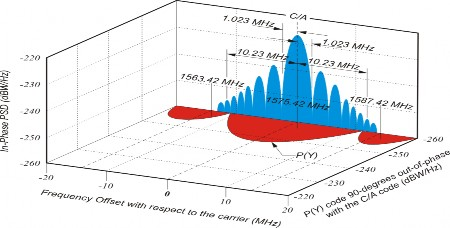Figure 1.1 GPS signal C/A and P(Y) code spread spectrum characteristic

L1 and L2 signal generation block diagram is depicted in Figure 1.2. Regarding  at the bottom left the data generator generates the navigation data. The code generators and the data generator are synchronized through the X1 signal supplied by the P(Y) code generator. After C/A and P(Y) code generated, the codes are combined with the navigation data through modulo-2 adders. The exclusive OR operation is used. The C/A code data and the P(Y) code data signals are supplied to the two modulators for the L1 frequency. The signals are modulated onto the carrier signal using the binary phase shift keying (BPSK) method.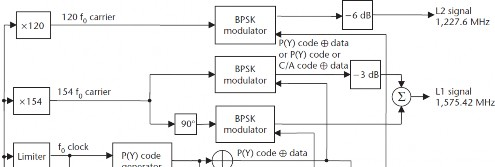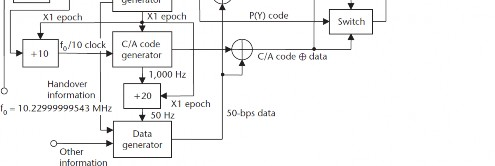Figure 1.2 L1, L2 signal generation block diagram 

The signal generated at the output can be described as:

s𝑘(t) = √2𝑃𝐶 (C𝑘(t) ⊕ D𝑘(t))cos(2πf𝐿1t) + √2𝑃𝑃𝐿1(P𝑘(t)

⊕ D𝑘(𝑡)sin(2πfL1t) + √2𝑃𝑃𝐿2(P𝑘(t) ⊕ D𝑘(t) sin(2𝜋𝑓𝐿2𝑡)

(1.4)

where, PC, PPL1, and PPL2 are the signal power of C/A code, P code (L1) and P code (L2) respectively. C𝑘 Is the C/A code sequence assigned to satellite number k, P𝑘 Is the P(Y) code sequence assigned to satellite number k, D𝑘 Is the navigation data sequence and 𝑓𝐿1 And 𝑓𝐿2 are the carrier frequencies of L1 and L2, respectively.

3. #### GNSS Receiver, Antennas and Front Ends

The ability to distinguish GNSS receiver components and units are crucial and help us classify the interference techniques in the next chapters. As depicted in Figure 1.3, a generic block diagram for GNSS receiver is presented. Stage 5 is correlation step that plays a key role in classification of detection and mitigation techniques. Pre-correlation and Post-correlation techniques alluded the place where techniques are take place. Now we will introduce each step to get an insight on GNSS receiver architecture.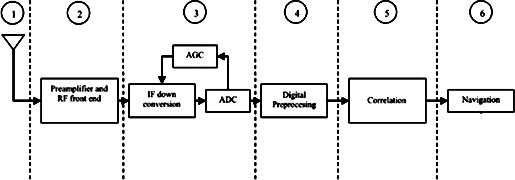Figure 1.3 Generic block diagram for GNSS receivers

First stage in a GNSS receiver is antenna, when we talk about antenna we should consider three fundamental parameters, Frequency/bandwidth, Polarization and gain pattern. Usually in L1 or 1575.42 MHz band all frontend components are in 50Ω of impedance and they divide into two type antennas, Active and Passive. A passive antenna is used in those designs with analog amplifier design in their receiver. Ordinary handheld devices that have GNSS receivers usually use an active

antenna, these antennas considered as an active element and requires power for the internal amplifier. By using bias-tree we can reach that aim, this component injects DC power onto the antenna cable to activate amplifier within the antenna.

Preamplifiers are unlike most of the filters an active components and that means they need power to accomplish their functions. As we talk about real non ideal amplifiers, amplification is not only on signal amplitude but also noise in resulting signal. The goal of using amplifier in this structure is to raise the extremely weak incident signal to a level that is acceptable for analog to digital conversion. Thus the amount of amplification is based on the specific ADC we manage to use in the receiver.

Analog to Digital Converter (ADC) is responsible for the conversion of the analog signal to digital samples. In order to choose proper ADC it is necessary to consider major parameters like number of bits, the maximum sampling frequency, the analog input bandwidth and analog input range. With respect to our requirements there are wide variety of ADCs available in the market. For example in case of GNSS and its modulation scheme and CDMA nature, ADCs with very little dynamic range from sampled signal could be an option. It has been shown that if single bit sampling is used then front ends degradation in the resulting process is less than 2 dB therefore, it is important to know if multi bit sampling is used or not, if yes then we need some form of gain control to provide proper quantization.

Digital preprocessing stage is responsible for sample preprocessing before an actual correlation takes place. Filters might be applies to ensure an optimum tracking performance and the noise floor usually needs to be determined. Just to get an insight for following chapters, most of detection and mitigation techniques that we are going to present later are implemented in this unit.

Correlator unit is the key operation unit for GNSS receivers to synchronize local signal with the incoming signal, generate GNSS observables, and retrieve the navigation message that will be used to provide a navigation solution for following navigation unit.

Navigation unit is responsible for finding the accurate position and velocity of the user. After the signal is finally acquired and tracked the computation of user position and velocity starts with respect to receiver reference clock.

1. ### Acquisition and Tracking

The purpose of acquisition phase stage is to identify all visible satellites to the user and gives a coarse estimate of signal parameters then more accurate estimation of signal parameters is performed in the tracking phase. We can say the main purpose of tracking is to refine the coarse values of code phase and frequency and keep track of these parameters. These two parameters initially determined during acquisition stage and then feed into tracking program. Carrier

frequency/phase code tracking implemented by help of Delay Lock Loop (DLL). In DLL three local replica generated and correlated with incoming signal, these three replica referred to as the early, prompt and late replica which separated with a half chip length. The same procedure happens for Doppler frequency tracking through Phase Lock Loop (PLL) filter. Both user and satellite movement can cause a phenomenon called Doppler frequency shift. In high user velocity it can reach up to 10 KHz but for stationary receiver on earth it will never exceed 5 KHz. The final code phase that extracted from this stage is needed for cross correlation between received signal and C/A code corresponding to specific satellite. This procedure eliminate signals from other satellites too. Figure 1.4 present a GNSS receiver channel stages from acquisition to Pseudorange calculation.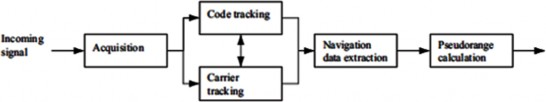Figure 1.4 One receiver channel. Acquisition block gives an approximate estimate of signal parameters. These parameters are refined by the two tracking blocks. After tracking, the navigation data can be extracted and pseudoranges can be computed

Acquisition procedure is similar to searching for each of the 1023 different code phases and test them by looking at the result coming out of this crossing. Figure 1.5 shows an example of acquisition for PRN32 while significant peak signal originating from PRN32. If the value exceed the specific threshold the satellite will acquired with the corresponding frequency and phase shift. The peak location in the Figure gives the parameters i.e. Doppler frequency and code delay we were looking for in acquisition stage.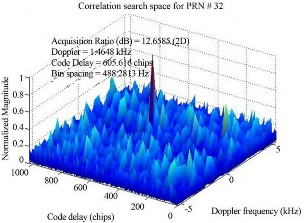Figure 1.5 Acquisition example plot for PRN32

After acquisition and tracking procedures finished and parameters extracted, eventually the C/A code and carrier wave can be removed from the signal and only the navigation data bits remain in navigation unit. After reading about 30s of data, the beginning of a subframe must be found in order to find the time when the data was transmitted from the satellite. When the time of transmission is found, the ephemeris data for the satellite must be decoded. Figure 1.6 shows navigation data structure. It has 1500 bits long frame containing five sub frames of 300 bits. One sub frame has a duration of 6 seconds and contains 10 words, each word with length of 30 bits. Ephemeris data (satellite position and velocity) packed in on to three sub frames and are repeated in each frame every 30s.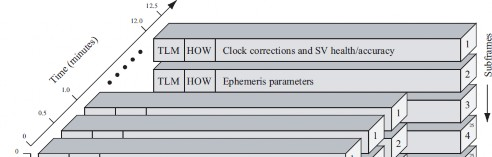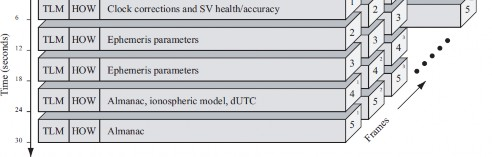Figure 1.6 Navigation data structure, Subframes 1, 2, and 3 repeat every 30 s while subframes 4 and 5 have 25 versions before repeating. That is, the entire navigation message repeats after

12.5 minutes. 

As it is obvious in Figure 1.6, sub frame four and five contain the almanac data, that repeated every 25 frames which means one entire navigation message lasts for 12.5 minutes. Almanac data is course orbital parameters for all satellites. Each satellite broadcasts Almanac data for all satellites. This Almanac data is not very precise and is considered valid for up to several months. Ephemeris data by comparison is very precise orbital and clock correction for each satellite and is necessary for precise positioning. Each satellite broadcasts only its own Ephemeris data. This data is only considered valid for about 30 minutes. Each word in each sub frame contains 6 bits for parity check and two words of each sub frame called TeLemetry and Hand Over Word (TLM, HOW). TLM

which is a first word in each subframe contains 8-bit preamble used for frame synchronization, HOW by the way contains subframe ID to show in which of the five subframes in the current frame this HOW is located.

3. ### Pseudorange and User Position Computation

As depicted in Figure 1.7, two spheres intercept to make a circle, the circle intersect with another sphere produce two points. Determination of user position need the third satellite but the ambiguity can be solve if user situated on earth’s surface. Unfortunately the description mentioned is oversimplification, this method of triangulation requires the receiver to know exact and precise time that the signal was transmitted and received. Even though time at the satellite 𝑡0 is known precisely due to atomic clock on board but the time at the receiver point 𝑡𝑟 is not known since the receiver internal clock is not precise. To fix the positioning accuracy to meter, it requires the GPS receiver to measure time accurately to 10-10 of a second. If companies who make GPS receivers wanted to use atomic clock in every GPS receiver, then it needs several thousands of dollars per unit, therefore you cannot see them in casual hand set devices. These inaccuracies in timing make huge error margins in calculated positions, therefore additional measurement can refine the position. The internal clock of the receiver measures 𝑡𝑟 incorrectly for all satellites and since this error offset is equal for all the satellites then using additional satellite can bring the position to one location.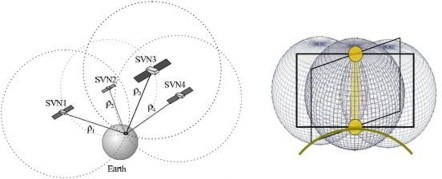Figure 1.7 the basic principle of GNSS positioning. With known position of four satellites and signal travel distance ρi, the user position can be computed.

The assumption of three satellite can simplify the calculation toward user position therefore in this dissertation we only consider three satellite positioning. Regarding  below basic equations gives the user position:

ρ1 = √(𝑥1 − 𝑥𝑢)2 + (𝑦1 − 𝑦𝑢)2 + (𝑧1 − 𝑧𝑢)2

{ρ2 = √(𝑥2 − 𝑥𝑢)2 + (𝑦2 − 𝑦𝑢)2 + (𝑧2 − 𝑧𝑢)2 ρ3 = √(𝑥3 − 𝑥𝑢)2 + (𝑦3 − 𝑦𝑢)2 + (𝑧3 − 𝑧𝑢)2

(1.2)

By measuring the time of the signal traveling from the satellite to the user the distance between the user and the satellite can be found. In the following we explain this distance measurement.

In order to calculate user position accurately, pseudorange measurement is necessary. This measurement is computed as the travel time from the satellite to the receiver multiplied by the speed of light in vacuum. The receiver has to estimate exactly when the start of a frame arrives at the receiver. This is done by adding the code phase to the time when the frame entered the receiver. When a satellite transmits at time instance 𝑡𝑠𝑖, the receiver time reception is 𝑡𝑢 and the distance between the satellite and user is:

ρiT = 𝑐(𝑡𝑢− 𝑡𝑠𝑖) (1.3)

Where c is the speed of light and ρT is the Pseudorange, 𝑡𝑠 true time of transmission from satellite i, 𝑡𝑢 is the true time of signal reception. Due to errors involving receiver clock time we can rewrite previous formula with other considered parameters in the following manner :

𝑖

ρ = ρ𝑘 + ΔD – c(𝛥𝑏 − 𝑏𝑢𝑡) + c(ΔT + ΔI + v + Δv) (1.4)

ΔD is the satellite position error effect on range, Δb is the satellite clock error, 𝑏𝑢𝑡 is the user clock bias error, ΔT is the tropospheric delay error, ΔI the ionosphere delay error, v is the receiver measurement noise error and Δv relativistic time correction.

Some of those errors can be corrected through received information but yhe user clock error cannot be corrected therefore, equation 1.2 must be modified as:

ρ1 = √(𝑥1 − 𝑥𝑢)2 + (𝑦1 − 𝑦𝑢)2 + (𝑧1 − 𝑧𝑢)2 + 𝑐𝑏𝑢𝑡

{ρ2 = √(𝑥2 − 𝑥𝑢)2 + (𝑦2 − 𝑦𝑢)2 + (𝑧2 − 𝑧𝑢)2 + 𝑐𝑏𝑢𝑡 ρ3 = √(𝑥3 − 𝑥𝑢)2 + (𝑦3 − 𝑦𝑢)2 + (𝑧3 − 𝑧𝑢)2 + 𝑐𝑏𝑢𝑡

(1.5)

Four equations are needed to solve for four unknowns 𝑥𝑢, 𝑦𝑢, 𝑧𝑢 and 𝑏𝑢𝑡, but these equations are difficult to solve since they are nonlinear equations. One common way is to linearize them. This aim is reachable by using least-squares method  which its description is not in the scale of this dissertation however, after using least squares method for linearization we can compute the user position with known parameters extracted from solved linear equations respectively.

# Interference in GNSS

Every communication system including GNSS receivers are suffering from interference during their operation however, GNSS signal specifically has weak power level (e.g., -160dBW) of the received signals, this level of power is even below noise floor and make GNSS signals vulnerable to any interference coming from transmitters close to receiver. Interference signals can be categorized based on their signal spectrum characteristics. They can divide to Narrow Band (NB) or Wide Band (WB) interference. The following subsection describes all these interference sources in detail. The focus of this dissertation is jamming signals.

1. ### Interference classification

There are different interference classification in literature, WB, NB, intentional and unintentional as general concepts therefore, every interference source can be assigned to any of them. As an example jammers can be categorized in sense of WB and NB interference and also by purpose of their emission.

1. ### Wideband and narrowband interference

WB interference has a bandwidth with frequency band bounded by the points corresponding to 10dB below the highest radiated emission. Usually intentional Ultra WB devices at any point of time has a bandwidth equal to or greater than 500MHz. There are two common forms of UWB, one based on sending very short duration pulses to transmit information and another approach using multiple simultaneous carriers. Figure 2.1 shows the example of UWB effect on PRN search space in GPS and consequently loosing satellite acquisition and lock as described in previous chapter.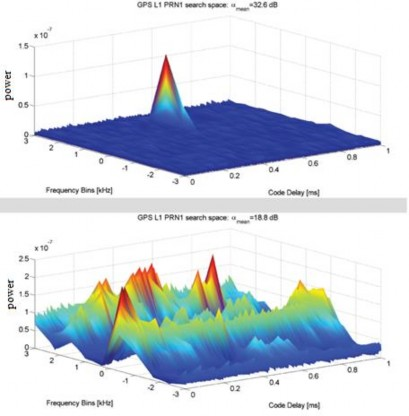Figure 2.1 Free interference environment versus in-band UWB interference environment in acquisition stage 

Narrow band interference has a small bandwidth while GNSS has C/A code bandwidth of 2MHz therefore, the spread spectrum properties of the GNSS signal enable the receiver to mitigate the influence of narrowband interference, however considering the characteristic of GPS C/A code with a short 1msec period, PRN sequence repeats every millisecond and corresponds to 1KHz space in spectrum, therefore a Continues Wave narrow band interference can mix with a strong C/A code line, leak through the correlator and degrade the C/N0. Figure 2.2 depict the simple CW interference on 1575.42MHz L1 band we measured in the laboratory.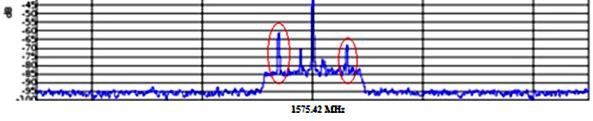Figure 2.2 Narrow band CW interference in L1 GPS band

2. ### Jamming interference

Another interference type we are going to concentrate in this dissertation is jamming, which is generally powerful signal over receiver operating frequencies and this type of interference includes continues wave (CW) and Chirp and Pulsed signals. Again each type of jamming can also classify in sense of NB and WB.

One example of jamming is Pulsed interference, Also can be known as an unintentional interference on GNSS systems since it primary originates from radars with Distance Measurement Equipment (DME) and Tactical Air Navigation (TACAN) signals, which are considered to have the most severe effects on GNSS in the L5/E5a band since they have high peak powers disturbing those bands. The main purpose of DME/TACAN, is distance measurement between an airplane and a ground station, this measurement works as follows: The aircraft transmits a pulse pair to the ground station which responds with a new pulse pair. The time it takes from receiving the pulse pair to transmitting the new one to the ground station is fixed to a certain value. This enables the interrogating aircraft to calculate the traveled time of the signal by subtracting this fixed delay and dividing by two. Pulsed pair used because of the greater resistance to background noise in contrast to using only one pulse. Although this interference usually happens unintentionally but it could be intentional too. Other unintentional interference examples are radio signals from cell towers, digital video broadcasting (DVB), Wi-Fi, Bluetooth, which has less sever effect on GNSS receivers rather than other interferences we are going to mention in this chapter.

3. ### Spoofing interference

More complicated interference exist in GNSS literature called spoofing . Simplistic attack via GNSS signal simulator is one of the easiest way of spoofing attack despite its costs that can reach up to \$400K or short period access renting of \$1K per week. The simulator starts making fake signal similar to authentic one without synchronization, consequently this type of interference is easy to detect, unsynchronized attack will act like jamming and cause user to lose the lock and redo acquisition step again simultaneously. Although this type of spoofing is easy to detect but it does not increase security since small change in spoofer can decrease probability of detection. This upgrade that makes simplistic spoofer to become more complicated one known as intermediate spoofing based on receiver, in this type of spoofer, receiver take place in spoofer and draw genuine GPS signals to estimate its own position, velocity and time. Based on these estimates, the receiver-spoofer generates counterfeit signals and start spoofing attack. Fortunately there is no commercial portable receiver based spoofer like simplistic one in the market, however recent Software Defined GNSS boards significantly increase this type of interference. Detecting this type of interference is very hard because receiver based spoofer synchronize its signals to GPS time and by configuring its direction to the target antenna align counterfeit and genuine signals as depicted in Figure 2.3.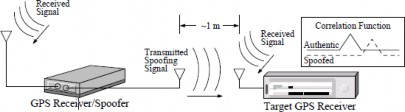Figure 2.3 intermediate spoofing attack via portable receiver spoofer 

The last type of spoofing called sophisticated attack via multiple phase locked portable receiver with receiving and transmitting antennas that are situated respectively on the upper and lower faces of the device and shielded to avoid self-spoofing. Now imagine several such devices sharing a common reference oscillator and communication link. Implementing this type of spoofers are very complicated and need phase coordinated structure which is also costly. The only method to countermeasure these type of spoofers is cryptographic authentications. Which is not in scale of this dissertation.

4. ### Meaconing interference

Meaconing is the interception and rebroadcast of navigation signals. They rebroadcast on the received frequency typically with power higher than the original signal to confuse enemy navigation. It usually has military usage which is not in the scale of this dissertation however, successful meaconing can cause aircrafts and drones to lure into ambush-ready zone in enemy airspace. Although there are some theories that 2010 Korean airline flight 007 is missing due to meaconing.

2. ### Example of jamming incidents

To get better sense of jamming threats and consequences we list some of incidents in below. According to  there are many jamming incidents happened purposely and accidentally in the recent years.

* Biskopstorp, Oct. 18 2011 -People reported that GPS and mobile phones did not work in southern Sweden. The police investigated the problem and found stolen boat, worth over one million SEK. Jammers were used to prevent the stolen property from being tracked.

*Newark airport, Aug. 3 2012: Technicians had been experiencing interference during pre-deployment testing of a ground-based augmentation system (GBAS). It was found that a Ford F-150 pickup truck was emanating radio signals within the restricted 1559 to 1610 MHz band. The

signals emanating from the vehicle were blocking the reception of GPS signals in the GBAS. The driver claimed that he installed and operated the jamming device in his company supplied vehicle to block the GPS-based vehicle tracking system that his employer installed in the vehicle.

*Newark airport, New Jersey, 2009: Engineers noticed that GPS receivers at Newark airport were suffering brief daily breaks in reception. It took two months for investigators from the Federal Aviation Authority to track down the problem, a driver who passed by on the nearby highway each day had a cheap GPS jammer in his truck to prevent his employer from tracking the vehicle.

*San Diego, 2007: Two navy ships in the San Diego harbor conducted a training exercise. To test procedures when communications were lost, technicians jammed radio signals. Unwittingly, they also blocked radio signals from GPS satellites. A big part of central San Diego was affected. The system for tracking incoming planes at the airport was malfunctioning, emergency pagers used for doctors at the Naval Medical Center stopped working, the traffic management system used for guiding boats in the harbor failed, about 150 base stations were malfunctioning and cellphones did not work, and bank customers trying to withdraw cash from local ATMs were refused.

There are many other instances for jamming accidents which we cannot mention here anymore. These events makes this threat vital for all civilian and military users.

3. ### Interference detection

The first step before mitigating interfering signal is to detect it, otherwise mitigation make no sense. During researches around detection techniques we occurred to two levels classification. Detection algorithms are happen in one of “pre-correlation” or “post-correlation” or both category, based on where their algorithm take the measurements along the receiver processing chain. Precorrelation algorithms make use of the digital signals at intermediate frequency (IF) or baseband and before the correlation process remove impairments common among all GNSS signals. Notch filtering and pulse blocking are examples of this kind. Unlike the post-correlation techniques the real time pre-correlation techniques are very computationally intensive. They will have to take snapshots of data from entire captured signal and it could be based on just a few second of data taken to estimates the histogram and power spectrum density (PSD). There are some test parameters like Fast Fourier Transform (FFT) size, evaluation window size that are all

configurable and should properly optimized during pre-correlation techniques. Figure 2.4 shows where pre correlation detection and mitigation techniques take place in a receiver processing chain.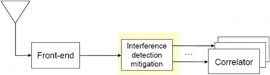Figure 2.4 Pre-correlation place inside processing chain

Post Correlation techniques implemented after correlation process while using the standard measurements such as satellite orbit information and signal to noise ratio (SNR). After signal correlated this method can be integrated within the acquisition and tracking unit too. Example of post correlation techniques is SNR detection or Cooperative Detection, which their implementation rely on the statistical tests of SNR and in Cooperative Detection case refers to having several detector devices / nodes that each collecting and testing their own data, and pooling the results for further analysis. In a well surveyed environment, the SNR measurements under nominal conditions from a static receiver are accurately predictable. A reference SNR value can be obtained via statistical curve fitting techniques based in a set of previously collected measurements such as transmission strength, atmosphere impact and environment geo-location. During online detection phase, each epoch of SNR measurements are compared to the thresholds and decision will be made respectively. Figure 2.5 depict where post correlation takes place.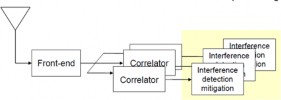Figure 2.5 Post-correlation place inside processing chain

1. ### Basics of detection theory

The concept of detection theory is a well-studied topic since many decades. In the following we will introduce some of basics in detection theory that is underlying step for better understanding of all methods that we are going to present in next chapters.

Hypothesis testing is the use of statistics to determine the probability that a given hypothesis is true and can perform in almost all stages of GNSS receiver (pre-post correlation, front-end,

measurement etc.). Equation 2.1 and 2.2 define hypothesis with respect to presence of received signal.

𝐻0: 𝑦[𝑛] = 𝑒[𝑛] (2.1)

𝐻0: 𝑦[𝑛] = 𝑥[𝑛] + e[n] (2.2)

Where n represents time, 𝑦[𝑛]is received sample signal, e[n] is the disturbance term embedding noise and interference and 𝑥[𝑛] is useful GNSS signal.

The comparison between test statistic and predetermined threshold can be formulated as:

𝐻1

<

Γ(𝑦) > η

𝐻0

(2.3)

where Γ(𝑦) is a test statistic and η is a predetermined threshold, clearly the fundamental problem of detector design is to choose the test statistic and set decision threshold to find the best performance.

Detection performance evaluate in term of Receiver Operating Characteristic (ROC), which gives probability of detection as a function of the probability of false alarm at a certain SNR.

𝑃𝑓𝑎 = Prob (𝜞(y) > η|𝐻0) (2.4)

𝑃𝑑 = Prob (𝜞(y) > η|𝐻1) (2.5)

There is always a tradeoff between detection probability and false alarm probability. That is, increased detection probability always comes at the cost of increased false-alarm probability and vice versa. This tradeoff is exactly what is determined by the ROC.

Detection algorithms are designed in two framework classical (deterministic) and Bayesian statistics. In the classical framework the objective is to choose test statistic Γ(𝑦) and threshold η to maximize 𝑃𝑑 subject to a constraint on 𝑃𝑓𝑎 < 𝛼. In Bayesian framework, by contrast, The

objective is to minimize the so called Bayesian cost and it is assumed that the source selects the true hypothesis at random according to some a priori probabilities presented as Pr (𝐻0) and Pr (𝐻1). Although there is difference in philosophy between those two approaches but both results in the same test form 2.3 where test statistic is the likelihood ratio :

Γ(y) = 𝑝(𝑦|𝐻1)

𝑝(𝑦|𝐻0)

(2.6)

Where (𝑦|𝐻0) and (𝑦|𝐻1) are probability density functions of the received signal 𝑦 under hypothesis 𝐻0 and 𝐻1 respectively

2. ### Energy detection

The whole concept behind energy detection is to measure the received signal energy during a finite time interval and comparing it to a predefined decision threshold. With respect to  and all basic concepts introduced in previous subsection the test statistic of the energy detector is:

1

Γ(𝑟𝑖) =

𝑁

∑|𝑟𝑖[n]|2

𝑁 𝑛=1 (2.7)

where 𝑵 is the number of samples. Figure 2.6 shows energy detection block diagram.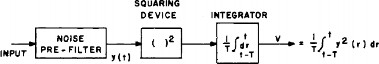Figure 2.6 Energy detection block diagram 

According to  a suitable sampling time window is chosen to satisfy the following constraint:

N ≥ T0B (2.8)

where T0 is the observational interval, and B denotes the band of interest. According to  the test static is approximated by a normal distribution 𝒩:

𝒩 (𝜎2,

2𝜎4

)

𝑁

(𝑟𝑖) ∼

𝒩 (𝑃 + 𝜎2,

{

2(𝑃 + 𝜎2)2

𝑁

) (2.9)

Where P is the received signal power and 𝜎2 is the noise variance which are the components of

𝑆𝑁𝑅 = 𝑃/𝜎2 (2.10)

The energy detection is characterized by the following theoretical probabilities of false alarm 𝑃𝑓𝑎 and missed detection 𝑃𝑚𝑑.

𝑃𝑓𝑎 = Prob(Γ(𝑟𝑖) > η|H0) > Q

lη − 𝜎2

2

𝗁 𝑁

𝜎2)

(2.11)

where Q represent the complementary cumulative density function of the standard normal distribution and η is the radiometer threshold. As a result, once fixed a target 𝑃𝑓𝑎fixed the test threshold can be computed by inverting the previous equation:

η = 2

𝑁

𝜎2 𝑄−1 (Pfa) + 𝜎2

(2.12)

where Q−1(. ) is the inverse of Q(.)

The energy detection method is widely used in many applications and has the ability to detect most of interference types and does not require knowledge of the interference source, however there is one drawback for this method and that is properly determination of decision threshold since the noise power is never perfectly known. Although in practice, thresholds descends from the test statistics of the jamming free data assessment and evaluation window. For example according to

 where a frequency domain detector was proposed for detection of a narrowband continues wave (CW), noise parameters (mean and variance of the power) were assumed to be estimated from an assessment window, in order to set a decision threshold. Test statics in this paper is based on the difference of estimated mean value of assessment window and evaluation window.

3. ### C/N0 based detection

The carrier-to-noise density (C/N0), i.e. the ratio of the useful power of a certain GNSS satellite signal to the noise power, this detection method based on the received signal quality from the corresponding satellite. As mentioned in classification subsection, this method is post-correlation method. In the pre-correlation techniques, components like antenna, AGC and ADCs have been used to detect and characterize the RFI and in post-correlation techniques, the observables of the receiver that are affected by RFI have usually been used to detect and characterize the interference. This characterization is done using the conventional definition of C/No as the squared mean of the correlator output divided by its variance. Estimates of the C/N0 are provided by commercial GNSS receivers and can be used for detection without requiring access to the internal signal processing in the GNSS chipset. Estimates of individual satellite C/N0 were used in  to characterize and detect a CW interference signal. In this work, the power of interference source is estimated by the value of AGC, which has relation to the background noise power. The main advantage of the C/N0-based detectors, just like the energy based detectors, is that they can be used to detect any type of interference signal. However, a serious disadvantage of these detectors is that they cannot distinguish between decreased GNSS signal powers and increased interference power. Therefore, this type of detectors is only practical in situations where the GNSS signal strength is always constant. For example, along roadsides we can use these detectors in fixed position to detect interferer, but they are not suitable in mobile scenarios in urban environments where the satellite signal strength varies because of shadowing caused by obstacles in the environment.

4. ### Detection Based on Antenna Array

Antennas has spatial properties that could help detecting interfering signal. Using multiple antennas in structure can distinguish jammer signal and authentic satellite signal for example in

 the authors exploiting the spatial properties of signals received from satellites and coming from different directions and a jammer respectively, then by analyzing the signals, the phase difference between two received antennas was estimated and used to detect interference signal. This phase difference shown by equation 2.10.

dφi = bT A 𝑠̂𝑖 + 𝑁𝑖 + B + 𝛾𝑖 (2.13) Where b is the baseline vector between the antennas in units of L1 cycles

A is a direction cosine matrix to rotate vectors in the east-north-up (ENU) frame to the body frame

𝑠̂𝑖 is the unit line of sight (LOS) vector if satellite i in the ENU frame Ni is an arbitrary integer ambiguity for satellite i

B is a constant “line bias” or time varying delta –clock term

𝛾𝑖 is the summation of all carrier phase error terms for satellite i

The plot in Figure 2.7 shows the basic idea of detection using multiple antennas. If dφi measurements that introduced above do not agree with the expected phase profiles within bounds set by the expected noise and attitude uncertainty then the jammer signal is detected. Regarding  algorithm, first the expected delta phases (dφi) are calculated, then for each satellite, error between the expected and the measured data is calculated and based on error threshold which is a function of satellite elevation, multipath and attitude uncertainty then a limited up-down counter is incremented or decremented. If the up-down counter reaches a specified maximum, it triggers an interference detection alarm. This method and algorithm can be defeated by “Sophisticated spoofer” which introduced in section 2.1.3.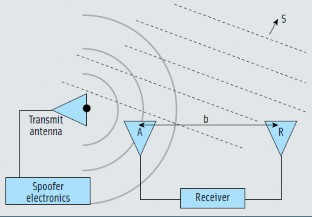Figure 2.7 Antenna diversity geometry for a single satellite and point transmitter 

As illustrated in Figure 2.8, an interferer transmitting from a single antenna has different geometry from those satellite tracked by receiver and distributed across the sky, so the deltas profiles for a point transmitter would all overlay each other except for contributions due to multipath and phase noise.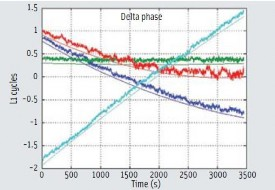Figure 2.8 Carriers phase deltas for four GPS satellites over one hour 

To conclude this type of detection we can say antenna arrays can be used not only to detect the jammer, but also to mitigate the effect of interference by beam forming and nulling techniques that we are going to describe later. An obvious drawback of these type methods is that it may be practically infeasible to have an antenna array on the typical platforms like smartphones and also high cost of implementation.

5. ### Detectors Based on GNSS/INS Integration

During navigation process if GNSS receivers combined and integrated with other sensors, detection can be performed by exploiting the integrated information of all sensors. Interference detection based on a combination of GNSS and an inertial navigation system (INS) was considered in . Regarding the paper an algorithm proposed in order to detect/compensate on-line interference effects when integrating the global navigation system and inertial navigation system (INS). Coupling the GNSS and INS usually performed by Extended KALMAN filter (EKF) which yield an accurate and robust localization. In this paper first they studied the impact of GNSS noise inflation on the covariance of the EKF outputs to compute a least mean square estimate of the potential variance jump. Second this estimation is used in Bayesian test which decides the interference are corrupting the GNSS signal or not. Other method proposed in this category is reflected in  where a secure reference receiver implemented and detector use this secure receiver to correlate the encrypted and unknown P(Y) code signals at both receivers. The detection method is rely on using the encrypted military Global Positioning System (GPS) P(Y) code on the L1 frequency in order to defend the publicly-known civilian GPS C/A code. Then detection of interference attacks is demonstrated by off-line processing of recorded RF data from narrowband

2.5 MHz RF front-ends, which attenuate the wideband P(Y) code by 5.5dB. The new technique can detect attacks using correlation intervals of 1.2s or less. Obviously, if information from multiple sensors is available, it should be beneficial to exploit this information for detection. Drawback of this method is that effective detectors could potentially become very complex, both in terms of the development, and also in terms of real-time computational complexity, but if implemented properly it could prevent threat effectively.

6. ### Other detection methods

There are various other type of interference detection methods mentioned in  as potential ways of discriminating an interferer signal from authentic GNSS signal that we list here:

1. Time-of-arrival discrimination

2. Amplitude discrimination

3. Polarization discrimination

4. Angle of arrival discrimination

5. Cryptographic authentication

7. Frequency switching

Each of these methods is suitable for specific type of interference. Techniques 1 and 2 could be implemented in software defined GPS receivers, but the techniques would be effective against only

the most simplistic spoofing attacks. Techniques 3 to 5 would be effective against some (but not all) more sophisticated attacks.

As an example (we will discuss with more details in next section) in case of using adaptive notch filters, this method can only be used in narrow band CW type of interference with respect to notch filter characteristics that pass all frequencies except those located in stop band or rejection band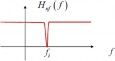Figure 2.9 Notch filter transfer function

Notch filter transfer function is Hnf ( f ) = {0 𝑓𝑜𝑟 𝑓 = 𝑓𝑖

1 𝑜𝑡ℎ𝑒𝑟𝑤𝑖𝑠𝑒

, There are several type of notch filter

which approximated with the ideal transfer function like Finite Impulse Response (FIR) , Infinite Impulse Response (IIR) , static, dynamic etc. We will discuss this method more in the next section.

7. ### Conclusion

At this subsection we can give a conclusion over detection methods introduced during this chapter. The comparison will be between each method complexity, strength or weak points.

 Detection Method Complexity Strength points Weaken points Energy detection Low -Can detect most type of interference -not require knowledge of jamming signal characteristic - decision threshold determination problem

 C/N0 detection Low -Can detect most type of interference - cannot distinguish between decreased GNSS signal powers and increased interference power -Good performance only in stationary with constant signal power Detectors Based on GNSS/INS integration High -Not only detect the interferer but also mitigate it - Not feasible for handheld devices implementation - very costly Other Detection Methods High Varies with respect to each one -complicated and costly

Table 2.1 Comparison between interference Detection methods

Some time-frequency domain techniques and spatial processing using antenna arrays are well suited for combating broadband jammers. Antenna arrays implementation is expensive and relatively large, so their applications are limited to fixed location GPS systems, or systems aboard ships or aircrafts. Another constraint is environment, for systems aboard aircraft it is not possible to adapt quickly to changing environment due to the speed of motion. For example in these cases short time Fourier transform (STFT) are suitable for such applications. a tradeoff between time and frequency must usually be made in time-frequency methods. Subspace processing and wavelet transforms usually produce better time-frequency resolution than STFT based methods

4. ### Interference mitigation

There are various methods to combat jamming in GNSS systems and mitigate their interference effects on receiver however, the choice of techniques depends on various factors such as space constraints, cost, power consumption and the environment where it takes place. Narrowband anti-jamming techniques include adaptive filtering, time-frequency methods, adaptive antennas and subspace processing. A short summary of each method is presented in the following subsections.

1. ### Adaptive filtering method (Notch Filtering)

Adaptive notch filters have been proven to be an effective solution for mitigating the impact of jamming and extending the operational capabilities of GNSS receivers. The notch filter is able to place a deep null with respect to the instantaneous frequency of a narrow band interference which is effective for narrowband jammers and can implement in applications that require low power consumption with small size package. The disadvantage of this technique is that it cannot be used when the jammer have unpredictable signal behavior. When the jammer has a predictable behavior, it is possible to subtract the predicted jammer signal from the received signal. Adaptive filtering techniques attempt to minimize the error involved in such a predictive filtering using techniques such as the Least Mean Squares algorithm.

Regarding  notch filtering have been widely used for the mitigation of CWI, the author of this paper do the experiment to use notch filter to mitigate jamming used typical in-car commercial jammer and results shows the threshold and its impact on GPS and Galileo systems before and after using Notch filter however, the analysis of its performance in the presence of a chirp signal is still missing in the literature but there is a suggestion in  to place multi stage Notch filter to countering high frequency variation scenarios. Cancellation algorithms are generally based on the interference cancellation principles. These algorithms start trying to estimate the interference from the most powerful coefficients of the transformed domain representation. The interfering signal is removed and the original signal is restored. When the signal is narrowband, Discrete Fourier Transform (DFT)-based frequency excision algorithms are particularly effective too. Despite their effectiveness, transform domain excision techniques are computationally intensive and other mitigation techniques have been considered in the literature. For example, notch filters are particularly effective for removing CWI.

Ordinary Notch filter has a Transfer function in z domain as:

1 − 𝑧0[𝑛]𝑧−1

(2.14)

𝛼

𝐻𝑛 ( 𝑧 ) = 1 − 𝑘

𝑧0

[𝑛]𝑧−1

Where 𝑘𝛼is the pole contraction factor and 𝑧0[𝑛] is the filter zero. 𝑘𝛼 Controls the width of the notch introduced by the filter whereas 𝑧0[𝑛] determines the notch center frequency. 𝑧0[𝑛] is progressively adapted using a Least Mean Squares (LMS) approach with the goal of minimizing the energy at the output of the filter.

Putting a deep null is possible and properly effective if we choose the zero adaptation parameters precisely therefore, we can track the interference frequency variations. It is possible to show that if the LMS algorithm used for updating 𝑧0[𝑛] then we are able to reach steady state:

Φ̂(𝑛𝑇 ) =  𝑓𝑠 ∠ 𝑧

(2.15)

[𝑛] ≈ Φ(𝑛𝑇 )

𝑠 2𝜋 0 𝑠

Which implies that 𝑧0[𝑛] can be used to estimate the instantaneous frequency of interfering signal ̂(𝑛𝑇𝑠)] , it is also presented that the magnitude of 𝑧0[𝑛] strongly depends on the amplitude of the interfering signal. Thus, |𝑧0[𝑛] | can be used to detect the interference presence and the notch filter can be used only if |𝑧0[𝑛]| passes a predefined threshold.

In conclusion, notch filtering disadvantages are the fact that these filters only works for predictable jammer signals, while choosing filter length and proper attenuation of these filters at the notch frequency varies in the adaptation and consequently a strong attenuation of the disturbing signal at notch filter is not guaranteed.

2. ### Wavelet based mitigation

Wavelet Transform Theory  is based on time-scaled mitigation method. This mitigation technique in the time-scale domain is based on the decomposition of a signal into its constituent components by using a local basis functions. These are obtained from a mother wavelet through scaled and shifted version. Hence, the original signal can be decomposed by linear combinations of a basis functions (wavelets) which localized in time and frequency domains. The basic idea is to provide detection and mitigation in the wavelet domain defining a threshold in each scale of the Wavelet decomposition, and then isolating the scale affected by the interference. In this way, both detection and mitigation can be applied only in a limited number of blocks. Continues wavelet transformation with a time function f(t) is:

𝛾 ( s, τ ) = ∫ 𝑓(𝑡) 𝜓

−∞

s,τ

(𝑡)𝑑𝑡

(2.16)

s,τ

Where * denotes the complex conjugation and 𝜓 (𝑡) is called the wavelet with its variables scale

𝑠 and translation 𝜏. The wavelet functions are deduced from one single wavelet, the so-called mother wavelet, by scaling and translation according to:

𝜓s,τ

1

(𝑡) =

𝑠

𝑡 − τ (2.17)

𝜓 ( )

𝑠

Where 𝑠 represents the scaling and 𝜏 the translation. Discrete Wavelet Transformation is needed when you working with discrete sampled data. The discrete wavelet function according to  is given by:

𝜓j,k

1

(𝑡) =

√𝑗𝑠0

𝑡 − 𝑘τ0𝑗s0 (2.18)

𝜓 ( )

𝑗s0

Where with integers j and k, discrete scaling steps s0 and time dilations τ0 but continuous with respect to the time axis t. It can be obvious that Wavelet functions give a band-pass like spectrum. With the fact that a compression in time can also be expressed as a stretch in the frequency domain together with a shifting up to higher frequencies. This can be happen to construct a series of Wavelets that cover the whole frequency range of our input signal where special attention needs to be paid that the stretched wavelet spectra touch each and intervene other’s neighbors as it can be seen in Figure 2.10.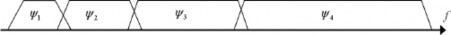Figure 2.10 Wavelet method spectra 

Practically it is impossible to cover all spectrum of an input signal. The solution is in applicable Wavelets is to stop the Wavelet stretching once the open hole is small enough and to fill it with a so called “scaling function”, that can be interpreted as a low-pass filter.

Now we move on to wavelet based mitigation algorithms for narrow band interference. The first step of this mitigation algorithm is the Wavelet decomposition of the incoming GNSS signal as described above. This decomposition has been achieved through the use of orthogonal local basis

function derived from the mother Wavelet. The last stage of this Wavelet based algorithm is the interference excision. Once the interference frequency components are identified and isolated, a synthetic reconstruction and correction of the interference signal in the time domain is provided below, performing an inverse Wavelet Transformation. In this way, it is possible to subtract this reconstructed interference signal from the original interfered signal, obtaining a new signal without the interference frequency component. This reconstruction procedure depicted in Figure 2.11.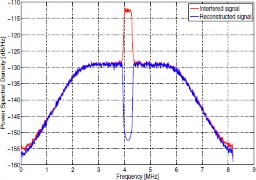Figure 2.11 Comparison between the Received Interfered Signal and the Signal at the output of the exciter 

Figure 2.12 represent the search space after the interference excision algorithm mentioned above is applied , it is possible to clearly observe a reduction of the noise floor, which allows the acquisition scheme to better detect the received signal and consequently successfully accomplish search and lock procedure. This analysis on the search space has been also carried out considering different interference scenarios according to the chosen interference carrier frequency offset with respect to the L1 GPS carrier in mentioned paper.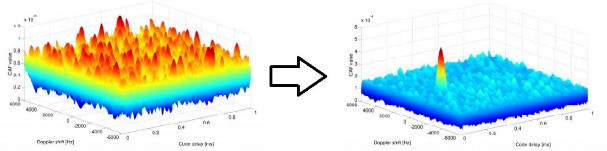Figure 2.12 Searching space before and after wavelet mitigation excision applied 

Wavelet mitigation method can also make significant improvement in tracking stage of GNSS receiver, In the ideal case, where the interference frequency components are identified and isolated by only one band of the Wavelet decomposition ,even for high power narrow-band interference it is still effective. Wavelet mitigation technique has a major drawback while in real conditions, the GNSS receivers have not any information about the interference signal which is impacting the quality of the GNSS signal, in particular, the power, the carrier frequency and the bandwidth of the jammer are unknown. Therefore, it is possible that the interference frequency components, which are unknown, could fall in different bands and then make the method useless.

3. ### Transformed domain mitigation

In this subsection we present incoming signal in another domain where the interference can be better identified and removed regarding . Description of this method and its complexity needed when they are used to detect and remove pulsed interference. In particular, the Karhunen-Loève Transform (KLT) is introduced and a preliminary assessment of its performance for pulsed interference mitigation is provided. The Karhunen-Loève Transform (KLT) characteristic provide the possibility to distinguish weak RF signals specifically detect very weak received signals hidden in noise in the framework of the SETI (Search for Extra Terrestrial Intelligence) program. However this technique can be extended to the GNSS scenario and offers several advantages with respect to the FFT approach. This domain gives us first the ability to work either with narrowband and wideband signals, second the flexibility in the choice of the basis functions, third the ability to merge a deterministic and a stochastic analysis of the signal and finally the capability to detect much weaker signals in comparison with other methods. The basic idea behind this technique is that the decomposition of the signal using Eigen functions in a vectorial space, which can have any form and they are orthonormal, and therefore can better adapt to the signal being processed and increase the detection performance. The KLT decomposition of a general time dependent function is:

X (t) = ∑ 𝑍𝑛𝑛(𝑡)

𝑛=1

(2.19)

Where 𝑍𝑛 are random scalar variables and 𝑛(𝑡) are the Eigen functions. The decomposition starts with the estimation of the linear auto-correlation function of the signal, Afterwards the Toeplitz matrix is computed and a set of eigen functions with their corresponding eigenvalues are extracted by solving linear equations. (In linear algebra, a Toeplitz matrix or diagonal-constant matrix, named after Otto Toeplitz, is a square matrix in which each descending diagonal from left to right is constant). It can be proved that a signal containing only noise is characterized by KLT small eigenvalues. Therefore, the presence of higher eigenvalues is a proof of the existence of

deterministic signals buried in the noise. Indeed, the greater benefit of the KLT transform is the capability to successfully detect not only CWI but also narrowband, wideband and chirp interferers, which are usually hard to handle. Nevertheless, the biggest drawback of the KLT technique is the complexity and computational burden required to extract a very large number of eigenvalues and eigen functions. A possible improvement is given by the Bordered Autocorrelation Method (BAM-KLT), which in principle allows to reduce the complexity, despite limiting the performance of the detection.

The BAM-KLT algorithm has been adopted from above algorithm in order to detect and isolate the interference. As it has been previously described, the KLT is able to decompose the signals in eigenfunctions whose shape is strictly dependent to the autocorrelation properties of the interfered signal. In this way, it is possible to separate the stochastic components from the deterministic ones which actually represent the information relative to the interference. The interference excision is performed applying an inverse KLT by using only those eigenfunctions representing the useful GNSS signal. The proper threshold in the eigenvalues domain has been chosen such that about 90% of the total eigenvalues are used for the GNSS signal reconstruction. This KLT based method is effective in detecting pulsed interference due to the clear features of autocorrelation of the interfering signals. The major issues derive from the computational complexity which is really high, for example, to Wavelet based methods. The ability of detecting and mitigating the signal is demonstrated by Figure 2.13 where the spectra of the interfered signal and of the signal after the KLT-based mitigation are compared. Distance Measuring Equipment (DME) pulses, those mentioned in interference classification in the beginning of the chapter is used as interferer in this experiment.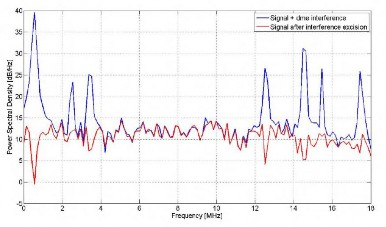Figure 2.13 Comparison before and after mitigation excitation (pulsed interferer by DME) 

The benefits for the GNSS receiver are further demonstrated by the advantages in the acquisition phase, where the ratio α between the highest correlation peak and mean value of the correlation floor has been considered as a figure of merit. Figure 2.14 shows how the noise floor of the

acquisition search space makes hard the detection of the correlation peak (α about 3dB). The KLT based mitigation significantly increases the separation of the correct peak from the noise floor. Thus, the KLT mitigation not only improves the spectral features of the signal wiping off the spurious components due to the pulsed interference, but it has also the remarkable advantage of preserving the integrity of the useful signal.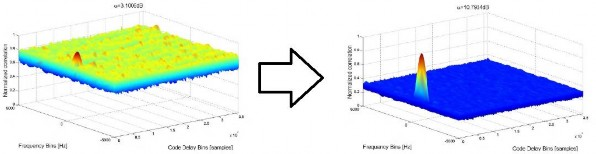Figure 2.14 compare acquisition space after and before mitigation excitation

As we showed during this subsection the high capability of the KLT based algorithm in detecting and extracting pulsed interference, while saving most of the energy of the received GNSS signal. Although for better understanding we can compare two method of Wavelet transform and Karhunen-Loéve Transform to get better in sight. Both methods give very good performance to mitigate interference but In particular implementing the Wavelet based mitigation technique needs a number of operations that depends on the number of GNSS signal samples N, on the number of Wavelet decomposition stage k, and on the length L of the Wavelet filter employed. Thus it offers the best trade-off between achieved performance in interference mitigation and number of operations required for the implementations, unlike the KLT based algorithm which results to be significantly more complex.

4. ### Subspace processing

Signals with rapid time-frequency varying characteristics can be the preferred at different transmitters or generated as a consequence of moving targets and scatters of fixed-frequency emitters. These signals are difficult to analyze, track, and remove using conventional methods. Recent developments in time-frequency (TF) signal representations have allowed accurate estimations of the signal TF signature which leads to effective suppression of undesired nonstationary signals. Regarding  they propose the use of time-frequency distribution (TFD) signal synthesis methods to estimate the jammer subspace of a general class of nonstationary jammers, provided that they are localizable in the time-frequency (t-f) domain. This method confines the jammer to a single dimensional subspace and, as such, reduces the GPS signal

distortion due to projections. The received signal is a mixture of the jammers, the additive noise, and the desired GPS signals. Therefore, in order to synthesize the jammer components, it is important to construct a mask in the t-f domain such that the masked t-f region contains most of the jammer energy and minimal GPS signal power.

This method uses the Extended Discrete-Time Wigner Distribution (EDTWD) to obtain a time-frequency distribution of the received signal. A received signal represented as :

𝐽

𝑥 = 𝑝 + ∑ 𝑗𝑣 + w

𝑣=1

(2.20)

Where x is a received signal [x(1),x(2),…,x(L)], and p is the GPS signal p = [p(1),p(2), … , p(L)],

𝑗𝑣 is the v’th jammer (v=1,…,J) , 𝑗𝑣 = [𝑗𝑣(1), 𝑗𝑣(2), … , 𝑗𝑣(L)] and w is the additive noise w=[w(1),w(2),…, w(L)]

Wigner distribution for a continuous received signal x(t) is defined as :

𝑊𝑥(𝑡, 𝑓) ≜ ∫ 𝑥 (𝑡 +

𝜏) 𝑥 (𝑡 −

𝜏) 𝑒−𝑗2𝜋𝑓𝑡𝑑𝜏

𝜏 2 2

(2.21)

The advantage of using the EDTWD in signal synthesis lies in the fact that it does not require a priori knowledge of the source waveform, and thereby avoids the problem of matching the two ”uncoupled” even-indexed and odd-indexed vectors. The overall procedure of EDTWD-based signal synthesis is summarized in the following steps:

1. Compute the EDTWD of the received signal and call it Wxx(t , f)

2. Take inverse Fourier transform of Wxx(t , f)

3. Construct the matrix Q = [ qi,j ]

𝑞𝑖,𝑗 = p (

𝑖 + 𝑗

, 𝑖 − 𝑗)

2

(2.22)

4. Take the Hermitian component QH of Q

5. Apply eigen decomposition to the matrix QH and obtain the maximum eigenvalue λmax and the associated eigenvector u

It can be shown that for a single jammer, the EDTWD is given by:

Wxx(t, f) = Wpp(t, f) +Wjj(t, f) +Www(t, f)+ 2Re(Wpj(t, f) +Wpw(t, f) +Wjw(t, f)) (2.23)

With the exception of the auto term Wjj (t, f), all other auto and cross-terms, including the auto term of the GPS signal Wpp(t, f), spread over the entire t-f domain. Therefore, to synthesize the jammer signal, exclusive from the GPS signal, one need to only mask out the auto term of the jammer.

This method selects a threshold value for the EDTWD to create a mask to extract the jammer signal such that the extracted signal contains almost all of the jammer and none of the signal. This method can also be used when multiple jammer signals are present. A nonstationary jammer suppression technique for GPS receivers, based on time-frequency distributions (TFDs), is proposed. The selection of the t-f mask in capturing the jammer power is discussed. The selection of the mask threshold value has been investigated and they have also considered array processing techniques in the underlying problem, and the advantages have been demonstrated in terms of array gain, improved jammer estimation, and spatial selectivity. Another method based on Space-Time-Frequency domain in Galileo system in investigated in . Proposed technique attempts to remove the undesired signal by projecting the received signal into the subspace orthogonal to the interference subspace, which is designed based on the estimation of the interference signature in space, time, and frequency.

Adaptive Antennas use spatial filtering techniques to eliminate the jammer signal. Like adaptive filtering, these techniques try to optimize a cost function. These techniques can be adapted for both narrowband and wideband jammers, but have the drawback of not being able to handle a large number of jammers in multiple locations. In antenna arrays, at least two antennas are needed to eliminate the effect of jammers from one location, hence such arrays cannot eliminate jammers originating from more locations than the number of antennas presented. The two basic approaches to spatial filtering are Null Steering and Beam Forming.

According to  many beamforming/nulling algorithms are used to enhance signal quality. Interference suppression performance is commonly measured by the signal-to-interference-plus noise ratio (SINR) at the output of the adaptive antenna array.

1. ### Adaptive antennas (Null steering)

Null steering antenna suppresses interference signal without any prior knowledge  , and can effectively weaken the strong signal among the weak signals, while the signal of GPS satellite is actually very weak, and is buried in the thermal noise, where interference signal is generally above the thermal noise. Null steering antenna makes up the short coming of GPS signal effectively. Regarding ,  the principle of the null steering antenna for GPS receivers is given and specifically the algorithm implemented in Power Inversion (PI) and analyzed. The antennas in the array are weighted so that any particular signal can be nulled. Null steering constantly computes the weights in order to minimize the received energy level (power minimization). In effect, this technique attempts to steer the antenna away from the interference. The implementation principle of null steering antenna for GPS receivers is shown in Figure 2.15.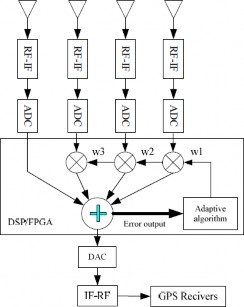Figure 2.15 Implementation principle of null-steering antenna for GNSS receivers 

The idea behind power minimization is that a beam is formed using the auxiliary elements and subtracted form the reference antenna. The beam formed using the auxiliary elements is computed as:

𝑁−1

𝑖

𝑦(𝑡) = − ∑ 𝑤𝑥𝑖(𝑡)

𝑖=1 (2.24)

The weights used to form the beams on the auxiliary elements are then determined by minimizing the mean square value of the difference between the reference antenna output and the output of the auxiliary beam:

𝑁−1 2

𝑖

𝑀𝑖𝑛𝑖𝑚𝑖𝑧𝑒 |𝑥0(𝑡) − 𝑦(𝑡)|2 = |𝑥0(𝑡) + ∑ 𝑤𝑥𝑖(𝑡)|

(2.25)

𝑖=1

𝑓𝑜𝑟 𝑤1, … , 𝑤𝑁−1 , Denoting x as the N×1 data vector: 𝑥𝑖(𝑡)=[𝑥0(𝑡), 𝑥1(𝑡), … , 𝑥𝑁−1(𝑡)]𝑇 while w is N×1 weight vector: 𝒘 = [𝑤0, 𝑤1, 𝑤2, 𝑤3]𝑇 Where 𝑤0=1 for the power minimization approach, the optimization problem dictating the optimal set of weights expressed as:

𝑀𝑖𝑛𝑖𝑚𝑖𝑧𝑒 |𝒘𝐻 𝒙(𝑡)|2 = 𝒘𝐻 𝑹𝑥𝑥 𝒘 (2.26)

For 𝒘 with subject to 𝒘𝐻 𝜹1 = 1 (2.27)

where 𝜹1 is the N ×1 vector 𝜹1 = [1,0, 0,0]𝑇 and we assumed wide sense stationarity over a small epoch of time such that 𝑹𝑥𝑥 is the 4× 4 spatial autocorrelation matrix.

𝑹𝑥𝑥 = 𝐸{𝑥(𝑡)𝑥𝐻(𝑡)} (2.28)

Using the method of Lagrange multipliers then:

𝐹(𝑤) = 𝒘𝐻 𝑹𝑥𝑥 𝒘 + 𝜆(𝒘𝐻 𝜹1 − 1) (2.29)

where 𝐹(𝑤) is Lagrange function and λ is Lagrange multiplier, the optimal set of weights vector is given by:

𝑤𝑜𝑝𝑡

1

= 𝛿 𝑇𝑅

−1𝛿

𝑅𝑥𝑥

−1𝛿1

(2.30)

1 𝑥𝑥 1

The optimal set of weights from a power minimization view point is thus proportional to the first column of the inverse of the autocorrelation matrix of array outputs.

According to  where power inversion used as iterative algorithm based on LMS then:

𝑤(𝑛 + 1) = 𝒘(𝑛) + 𝜇{𝑥0(𝑛) − 𝒘𝑥𝑎(𝑛)} 𝑥𝑎(𝑛) (2.31)

where 𝜇 is the step-size parameter or weighting constant. 𝑥𝑎(𝑛) = [𝑥1(𝑛), 𝑥2(𝑛), … , 𝑥𝑁−1(𝑛)]𝑇 is auxiliary antenna output. This method has the disadvantage of potentially reducing the signal level too. This technique is a pre-correlation technique and does not require any knowledge of the desired signal.

The core idea behind beamforming is using GNSS antenna motion to synthesize a virtual antenna array for GNSS spatial processing (beamforming and AOA estimation). Several classical beamforming techniques previously developed for a physical real array are applied to a synthetic array based system. Their performance is compared theoretically in terms of the antenna beamforming beam-pattern. Angle of arrival estimation for GNSS signals is successfully demonstrated with both simulated datasets from an advanced hardware simulator and field measurements .

Before explaining this mitigation technique we should define the concept of Synthetic array. Synthetic array processing is the transformation from the temporal samples received by a moving antenna to spatial samples. When an antenna is moving, the receiver receives signals with different phases at different times. If the propagation channel is stationary, this transformation is trivial. Assuming there are M real GNSS antennas located uniformly on a circle, signal samples are

collected about every 𝑡𝑠𝑦𝑛𝑡ℎ𝑒𝑡𝑖𝑐 seconds, the batch of samples collected by each antenna can be divided into M equal parts. If the channel is stationary during the data recording period, the signal blocks used for beamforming or AOA estimation can be asynchronous in time. A synthetic array uses this property to create a virtual antenna array. In reality, the propagation channel is not stationary, because GNSS satellites are not static, and satellite clocks and receiver clocks have different levels of drifts. Thus, the carrier phase of the received signal samples collected at different time and spatial points is due to not just the motion of the antenna but also the effect of satellite motion, satellite and receiver clock drifts. To successfully perform spatial processing (e.g. beamforming and AOA estimation), the phase/Doppler change due to satellite motion, satellite and receiver clock drifts need to be compensated prior to using the correlator outputs for beamforming. If the location of the antenna is exactly or approximately known, the satellite motion and satellite clock drift can be compensated with ephemeris information. An alternative approach is to use the Doppler estimates from a static reference antenna for satellite motion and satellite clock drift compensation (potential extension of Assisted GPS), which is similar to the concept of single differencing between the rover and the base in a GNSS navigation solution. The receiver clock drift can be further removed by differencing between two satellites from the same antenna.

Therefore, in conclusion here we will discuss the beamforming algorithms for synthetic arrays which is as same as physical arrays algorithm with the fact that compensation process should applied on them to remove phase changes due to satellite motion and satellite clock drifts with receiver clock. Several classical beamforming algorithms discussed in GNSS literature, however, these algorithms were developed for physical arrays, they can be applied to synthetic arrays after the compensation process, which removes phase changes due to satellite motion, satellite clock drifts and receiver clock drifts. In general, beamforming is achieved by multiplying the spatial correlators (at the post correlation level) or spatial signal samples (at the pre-correlation level) by a complex weight vector w. The algorithms described here are all linear therefore, they can be applied at the pre-correlation level or the post-correlation level depending on the application. In general, interference detection/mitigation is applied at the pre-correlation level, while multipath mitigation is performed at the post-correlation level.

Before we introduce Beamforming algorithms in this subsection we should introduce Signal model for synthetic arrays, therefore, as a starting point, it is assumed that there is only GNSS signal and white Gaussian noise in the received samples. At the post-correlation level, assuming the local PRN code is perfectly synchronized with the incoming signal, the prompt correlator is given as :

𝑃(𝑡, 𝑟𝑡) = 𝑎(𝑡, 𝑟𝑡)𝑑(𝑡)𝑒𝑥𝑝{ 𝑗𝛥𝜙(𝑡, 𝑟𝑡)} + 𝑛𝑝 (2.32)

𝛥𝜙(𝑡, 𝑟𝑡)} = 𝜙(𝑡, 𝑟𝑡)} – 𝜙̂ (𝑡 – 𝑇𝑐𝑜ℎ , 𝑟𝑡 – 𝑇𝑐𝑜ℎ) (2.33)

Where, 𝑟𝑡 is the position vector with respected to the reference point of the synthetic array (e.g. the center of the circular array) at epoch t, a is the signal amplitude, d is the navigation data, R is the PRN code correlation power, n is the white Gaussian noise, Δϕ is the carrier phase difference, Tcoh is the coherent integration time.

The navigation data and the carrier phase difference due to satellite motion and clock drifts need to be compensated. For post-correlation beamforming, since the carrier phase is tracked by the PLL in the baseband processor, back compensation of the correlator is required, which tries to restore the full carrier phase information back to the correlator so that all the signal information will be contained in the compensated correlator. Assuming the signal power does not change rapidly during the synthetic spatial processing, the compensated correlator is:

𝑘

𝜙(𝑡𝑘 , 𝑟𝑖 ) = ∑ 𝜙̂ (𝑡𝑖 ,𝑟𝑖)

𝑖=1 (2.34)

𝜙𝑐𝑜𝑚𝑝(𝑡0, 𝑟𝑘) = 𝜙(𝑡𝑘, 𝑟𝑘 )– 𝜙𝑟𝑒𝑓( 𝑡𝑘, 𝑟𝑘) (2.35)

𝑃𝑐𝑜𝑚𝑝(𝑡0, 𝑟𝑘) = 𝑎 exp{𝑗𝜙𝑐𝑜𝑚𝑝(𝑡0, 𝑟𝑘)} + 𝑛̅𝑝 (2.36)

where 𝜙(𝑡𝑘, 𝑟𝑘 ) is the total carrier phase due to antenna motion, satellite motion and clock drifts at epoch k, 𝜙̂ (𝑡𝑖 ,𝑟𝑖) is the estimated carrier phase from PLL at epoch i, 𝜙𝑟𝑒𝑓( 𝑡𝑘, 𝑟𝑘) is the carrier

phase due to satellite motionand clock drifts only at epoch k,

̅𝑛̅𝑝̅ is the noise after the

compensation, 𝜙𝑐𝑜𝑚𝑝(𝑡0, 𝑟𝑘)is the compensated carrier phase which is only due to antenna motion. Compensated correlators can be interpreted as representing the correlators from an antenna array. These “spatial correlators” can be rewritten in a matrix form and if we write that matrix as a function of the LOS signal array manifold vector v ( 𝑘𝑠 ) then:

exp{𝑗𝑘𝑇𝑟 }

𝑃𝑐𝑜𝑚𝑝(𝑡0) = 𝑠𝑝 𝑣(𝑘𝑠) = 𝑠𝑝

𝑠 0

.

.

.

+ 𝑛̅𝑝

(2.37)

[exp{𝑗𝑘𝑇𝑟 }]

𝑠 𝑁−1

sin 𝜃

2𝜋

cos 𝜃𝑐𝑜𝑠𝜑

𝑘𝑠 =

λ [cos 𝜃𝑠𝑖𝑛𝜑] (2.38)

Where 𝜃 is the elevation angle, 𝜑 is the azimuth angle, 𝑠𝑝 is signal at the reference point of the array. Now it is the time to introduce Beamforming algorithm. In general, beamforming is achieved by multiplying the spatial correlators (at the post correlation level) or spatial signal samples (at the pre-correlation level) by a complex weight vector w. The algorithms described here are all linear, therefore they can be applied at the pre-correlation level or the post-correlation level depending on the application. In general, interference detection/mitigation is applied at the pre-correlation level, while multipath mitigation is performed at the post-correlation level. Delay and sum beamforming is one of the fundamental beamformers and the optimum beamformer in white Gaussian noise, it can be considered as the spatial matched filter that can maximize the array signal-to-noise ratio (ASNR) in the white noise channel. The mathematical expression for the weighting vector of the Delay-and-sum beamformer is:

𝑤 =

1

𝑁 𝑣 (𝑘𝑠)

(2.39)

Where N is the number of virtual antennas in the array, 𝑣 (𝑘𝑠) is the true steering vector or array manifold vector of the LOS GNSS signal. Minimum Variance Distortion less Response (MVDR) and Minimum Power Distortionless Response (MPDR) Beamformer are optimum in both maximum likelihood and maximum Array Signal to Noise Ratio (ASNR) sense in correlated or uncorrelated Gaussian noise channels. Here, the term noise is meant in a generic sense, which includes interference, multipath and white noise. In fact, the MVDR beamformer can be viewed as the spatial generalized matched filter. The cost function of this beamformer is:

𝐹 = 𝑤𝐻 𝑅𝑛 𝑤 + λ [𝑤𝐻 𝑣 (𝑘𝑠) − 1 ] + λ[𝑤𝐻 𝑣 (𝑘𝑠) − 1] (2.40)

Where w is the array weighting vector, 𝑅𝑛 is the correlation matrix of the unwanted signals (noise, interference and multipath), 𝑣 (𝑘𝑠) is the true steering vector/array manifold vector. In practice, it is difficult to obtain 𝑅𝑛 from weight vector w, since the signal component is always embedded in the received measurements. Therefore, an alternative beamformer named MPDR was proposed. The cost function of the MPDR is defined:

𝐹 = 𝑤𝐻 𝑅𝑥 𝑤 + λ [𝑤𝐻 𝑣 (𝑘𝑠) − 1 ] + λ[𝑤𝐻 𝑣 (𝑘𝑠) − 1] (2.41)

Where the only difference is 𝑅𝑥 , which is a correlation matrix of the received samples (signal + noise + interference + multipath)

The weight vector (MPDR) will be defined as:

𝐻

𝑚𝑝𝑑𝑟

𝑣𝐻(𝑘𝑠) 𝑅−1

𝑣𝐻(𝑘𝑠) 𝑅−1 𝑣 (𝑘 )

𝑤 =

𝑥

𝑥 𝑠

(2.42)

If the AOA of the Line-Of-Sight (LOS) GNSS signal is known perfectly, the performance of MVDR and MPDR will be the same. However, the performance of MPDR degrades more rapidly than MVDR when the LOS AOA mismatch appears. One major difference between the Delay-and-sum beamformer and the MVDR/MPDR beamformer is that the MVDR/MPDR beamformer takes into account the interference and multipath in the channel. The inverse operation of the unwanted signal correlation matrix or received sample correlation (RnandRx) will tend to null out the interference and multipath signals. In other words, interference and multipath signals will be mitigated even if their AOAs are unknown, given that the unwanted signal correlation matrix 𝑅𝑛 is known precisely. In reality, 𝑅𝑛 must also be estimated. Therefore, MVDR and MPDR are theoretically optimum but not practically robust. It should be noted that forward-backward smoothing techniques should be applied for any spatial processing which needs to manipulate the correlation matrix (e.g. Inversion and eigen-decomposition), since multipath signals are coherent with the LOS signals.

Linear Constraint Minimum Variance/Linear Constraint Minimum Power (LCMV/LCMP Beamformer are the generalized versions of MVDR and MPDR. They allow multiple linear constraints in the cost function. The cost functions for LCMV and LCMP are shown below:

𝐽 = 𝑤𝐻 𝑅𝑛 𝑤 + λ [𝑤𝐻 𝐶 − g𝐻] + λ[𝐶𝐻 𝑤 − g] (2.43)

𝐽 = 𝑤𝐻 𝑅𝑥 𝑤 + λ [𝑤𝐻 𝐶 − g𝐻] + λ[𝐶𝐻 𝑤 − g] (2.44)

where w is the array weighting vector, 𝑅𝑛 is the correlation matrix of the unwanted signals (noise, interference and multipath). 𝑅𝑥 is the correlation matrixof the received samples (signal, noise, interference andmultipath). C is the constraint matrix that contains all the steering vectors used in the defined constraints. g is the constraint vector that contains all the numerical values for all the constraints. The weight vectors for LCMV and LCMP can be shown as:

𝑤𝐻 = g𝐻[C𝐻𝑅 −1

−1 𝐻

−1 (2.45)

𝑙𝑐𝑚𝑣

𝑛 C]

C 𝑅𝑛

𝑤𝐻 = g𝐻[C𝐻𝑅 −1

−1 𝐻

−1 (2.46)

𝑙𝑐𝑚𝑝

𝑥 C]

C 𝑅𝑥

The beamformers introduced above can be applied for multi-directional beamforming, although only one LOS steering vector was used in the equations above. For GNSS signals, there is only one desired signal after correlation because of the pseudo-orthogonal nature the spreading codes. At the post-correlation stage, beamforming will be performed in each PRN tracking channel. In other words, 12 beamformers are required for a 12 tracking channel GNSS receiver. Synthetic array beamforming can be performed at the precorrelation level as well. In that case, only one multi beamformer (beams pointing at all satellites in view) is required. The weight vectors for the MVDR/MPDR based the multi-beam antenna array are:

𝑅−1 𝑉 (𝑘 ) (2.47)

𝑤𝐻 = 𝑛

𝑠 𝐼

𝑛

𝑠

𝑚𝑣𝑑𝑟

𝑉𝐻(𝑘𝑠) 𝑅−1 𝑉 (𝑘 )

𝑅−1 𝑉 (𝑘 ) (2.48)

𝑤𝐻 = 𝑥

𝑠 𝐼

𝑥

𝑠

𝑚𝑝𝑑𝑟

𝑉𝐻(𝑘𝑠) 𝑅−1 𝑉(𝑘 )

Where w is the array weighting vector, 𝑅𝑛 is the unwanted signals (noise, interference and multipath)correlation matrix, 𝑅𝑥 is the received samples (signal ,noise, interference and multipath) correlation matrix, I is a N ×1 vector of ‘1’s, N is the number of synthetic antennas, 𝑉(𝑘𝑠)is the true steering vector/array manifold vector. Beamforming with a synthetic array can enhance the directivity of the antenna thus mitigating interference and multipath. One of the major requirements of beamforming is the array manifold vector. In other words, the trajectory of the motion and the AOA of the GNSS signals should be known. In this section, three algorithms for AOA estimation will be discussed briefly. The Beam Scan algorithm is one of the most classical AOA estimation methods. It estimates AOA by scanning the signal power at each possible angle of arrival and selects the one with the maximum power. The power from a particular direction is measured by first forming a beam in that direction and setting the beamformer weights equal to the array manifold vector corresponding to that particular direction. The mathematical formula for this technique is:

𝜃̂ = 𝑎𝑟𝑔𝜃 max P(𝜃 , 𝜑)

𝐻 ( )

2

= 𝑎𝑟𝑔𝜃 max {𝐸 {‖𝐯 (𝑘𝑎 𝜃 , 𝜑 )𝐱‖ }}

= 𝑎𝑟𝑔𝜃 max{𝐯𝐻(𝑘𝑎(𝜃 , 𝜑))𝑹𝑥𝐯(𝑘𝑎(𝜃 , 𝜑))}

(2.49)

(2.50)

(2.51)

̂𝜑

= 𝑎𝑟𝑔𝜑 max 𝑃(𝜃 , 𝜑)

(2.52)

where 𝜃̂ is the estimated elevation angle, 𝜑̂ is the estimated azimuth angle, 𝐯(𝑘𝑎(𝜃 , 𝜑) is the assumed array manifold vector at elevation 𝜃 and azimuth 𝜑, P(𝜃 , 𝜑) is the power at elevation 𝜃 and azimuth 𝜑, 𝐱 is the vector of received samples (raw samples or compensated correlator outputs) and 𝑹𝑥 is the sample correlation matrix.

Capon’s Algorithm Similar to the previous algorithm, Capon’s algorithm also estimates AOA by measuring the power of received signals in all possible directions. The major difference is the weight vector. Instead of using the assumed array manifold vector, it uses the weight from the MPDR beamformer based on the assumed direction. In other words, the power from the AOA estimate is measured by constraining the signal power gain to be unity from the assumed direction of arrivals and using the remaining degrees of freedom to minimize the contribution to the output power from all other directions. The mathematical formula is as follows:

𝑅−1 𝐯(𝑘

(𝜃 , 𝜑))

𝑤(𝜃 , 𝜑 ) =

𝑥 𝑎

𝑥

𝑎

𝑉𝐻(𝑘𝑎(𝜃 , 𝜑)) 𝑅−1 𝐯(𝑘

(𝜃 , 𝜑)) (2.53)

 𝜃̂ = 𝑎𝑟𝑔𝜃 max{𝐸 {‖𝐰𝐻(𝜃 , 𝜑)𝐱‖2}} (2.54) = 𝑎𝑟𝑔𝜃 max{𝐰𝐻(𝜃 , 𝜑) 𝑹𝑥𝐰(𝜃 , 𝜑)} (2.55) 𝜑̂ = 𝑎𝑟𝑔𝜑 max{𝐸 {‖𝐰𝐻(𝜃 , 𝜑)𝐱‖2}} (2.56) = 𝑎𝑟𝑔𝜑 max{𝐰𝐻(𝜃 , 𝜑) 𝑹𝑥𝐰(𝜃 , 𝜑)} (2.57) 𝜃̂ is the e stimated elevation angle, 𝜑̂ is the estimated azimuth angle, v(𝑘𝑎(𝜃 , 𝜑) is the

Where

assumed array manifold vector, x is the vector of received samples and 𝑅𝑥 is the sample correlation matrix.

The Multiple Signal Classification (MUSIC) algorithm belongs to the family of subspace algorithms, which can provide AOA or frequency estimates with super-resolution. It can be easily shown that the array manifold vectors corresponding to the incoming signals lie in the signal subspace and are therefore orthogonal to the noise subspace. One way to estimate AOAs is to search through the set of all possible directions and find those that are orthogonal to the noise subspace. Given the array manifold vector of the incoming signals 𝐯(𝑘𝑎(𝜃 , 𝜑)) and the matrix corresponding to the noise subspace Q𝑛 then below equation should be true:

𝐯𝐻(𝑘𝑠(𝜃 , 𝜑))𝐐𝑛 = 0 (2.58)

Therefore mathematical formula for MUSIC algorithm is:

𝜃̂ = 𝑎𝑟𝑔𝜃 max P𝑀𝑈𝑆𝐼𝐶(𝜃 , 𝜑 ) (2.59)

= 𝑎𝑟𝑔𝜃 max {𝑉𝐻(𝑘

(𝜃 , 𝜑))𝑸

1

𝑸𝐻 𝐯(𝑘

}

(𝜃 , 𝜑))

𝑎 𝑛 𝑛 𝑎

(2.60)

𝜑̂ = 𝑎𝑟𝑔𝜑 max P𝑀𝑈𝑆𝐼𝐶(𝜃 , 𝜑 ) (2.61)

= 𝑎𝑟𝑔𝜑 max {𝑉𝐻(𝑘

(𝜃 , 𝜑))𝑸

1

𝑸𝐻 𝐯(𝑘

}

(𝜃 , 𝜑))

𝑎 𝑛 𝑛 𝑎

(2.62)

Where 𝜃̂ is the estimated elevation angle, 𝜑̂ is the estimated azimuth angle, v(𝑘𝑎(𝜃 , 𝜑) is the assumed array manifold vector,𝑃(𝜃 , 𝜑) is the signal power, 𝑄𝑛 is the noise subspace matrix, which can be extracted from the sample correlation matrix. Although MUSIC algorithm theoretically provides AOA estimates with super-resolution, the correlation matrix and the number of the desired signals should be known. In practice, these parameters need to be estimated. The accuracy of these estimates will strongly affect the performance of MUSIC.

The AOA spectrum for Beam Scan, Capon and MUSIC are shown in Figure 2.16 for PRN 3.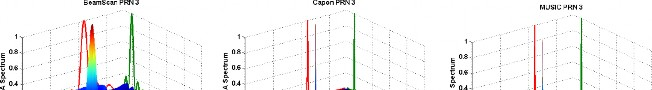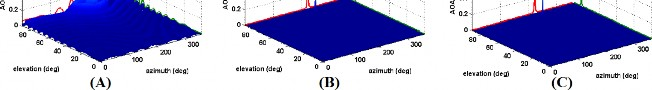Figure 2.16 AOA spectrum (A) BeamScan (B) Capon(C) MUSIC algorithm on PRN3 

### 2.4.6 Conclusion

At the end if we want to summarize all techniques in a table and compare them in parameter like Cost, Size, Power and Complexity we can see:

 Mitigation techniques: Cost Size Power Flexibility Complexity note Adaptive Filtering Low Small Low Only predictable behavior interferer not suitable for chirp signal Wavelet transform Low Small Low env , res Low Unknown jammer parameters could degrade performance Transformed domain - - High - High Mitigating narrowband, wideband and chirp interferers Subspace processing Low Small Low No need of a priori information of jammer Adaptive Antenna(Nul l steering) High Large High High Not required knowledge of desired signal Adaptive Antenna(Bea m forming) High Large High High

Table 2.2 Summery of studied jamming mitigation techniques

env = Flexible to changing the environment res = Flexible to resolution

Although we consider different techniques here individually but there are possibility of having combination of different techniques in order to robust the GNSS receiver against interference.

# Joint interference detection and localization

Regarding  a novel algorithm for detection and localization proposed by extracting data from a network of sensing nodes and feeding their data through an algorithm that uses Extended Kalman Filter (EKF) to reach that aim. The algorithm runs on a network of low-complexity sensor nodes estimating their relative distance from an interferer. In particular, we evaluate distances based energy measurements, then, detection phase starts by first EKF who is in charge of aggregate decision, when interference presence detected second EKF starts estimating jammer source location. In this following subsections we first briefly introduce some previous works on detection and localization and then describe the algorithm in detail.

1. ### Previous interference detection and localization overview

The question that may appear in mind is why we should propose a new algorithm for detection and localization? In order to answer this question we should take a look to previous works on this area and highlight their strength and weakness. In GNSS community the most effective techniques for interference detection and localization are based on Angle of Arrival (AOA), one common approach to obtain AOA measurements is to use an antenna array on each sensor node as described in previous chapter . However this technique should supported with a complex receivers equipped with multiple antennas or capable of digital beam forming which is very complex in implementation and very costly in general.

Another effective localization way is represented by Time Difference of Arrival (TDOA) estimation. Due to  The TDOA technique allows to estimate the delay of arrival between two stations. Three stations and three estimations are useful to estimate the X, Y, Z source position. TDOA has some major drawbacks including high data rate generated between each node in air medium since technique transmit a temporal part of the received signal and processing inefficiency in case of CW or narrow band signals.

Here we have a novel, low complexity algorithm for joint detection and localization of interfering source in GNSS which doesn’t have the same problems mentioned above. The algorithm runs on a network of sensor nodes estimating their relative distance from an interferer. In particular, we evaluate distances based energy measurements, but other common techniques may be adopted (e.g., received signal strength, correlation-based techniques). Once measurements are collected, an EKF is in charge of data fusion and jammer localization. As a result, this algorithm enables joint detection and localization of interference sources.

1. ### Multi site radar system

With respect to  Multi Site Radar Systems (MSRS) is well known as the earliest bi static continues wave radars. They detected an object as it crossed the transmitting station-receiving station baseline by measuring the beat frequency of its Doppler-shifted reflection and direct signal propagating from the transmitting station to the receiving station. After the invention of the duplexer at the US Naval Research Laboratory in 1936, which provided a means of using a common antenna for both transmitting and receiving, monostatic radars became practical and interest in bistatic radars became dormant. The interest in bistatic radars was revived again in the 1950s. If the target is not transmitting, it is necessary to highlight the target by transmitted signal. If the target is active, a transmitter is not required. In the case of active radar, one antenna performs both functions, transmitter and receiver. One of the first MSRS in the United States is the NAVSPASUR (Navy Space Surveillance System). It is a CW MSRS, in operation since 1960 that detects orbiting objects as they pass through the electronic “fence” over the continental United States. The large linear arrays of the transmitting stations generate stationary, vertical, coplanar fan beams, oriented along the great circle. The Multi static Measurement System (MMS) was deployed in 1978-1980 at the Kwajalein Missile Test Range (Marshall Islands) as a part of the Kiernan Re-entry Measurement Site (KREMS). The high-power Tradex L-band and Altair UHF radars at Roi-Namur Island are utilized to illuminate targets and to receive target echoes. All these systems have baselines on the order of at least tens or hundreds of kilometers. This type of localization is specifically for long baseline utilization in range of at least ten kilometer but we are looking for shorter baselines.

2. ### Passive localization

Passive Localization and Signal Processing is another reported localization technique. Most important result reported in the passive localization literature has to do with the canonical form of the maximum likelihood (ML) estimator of source location parameters given the received sensor signals. Regarding  that the ML estimate of source position is that which maximizes the power in the output of the filtered beamformer shown in Figure 3.1.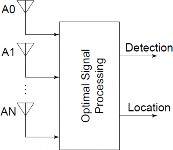Figure 3.1 Filtered beam former or step processing

Regarding ,  Hahn and Weinstein showed that, in the small-error region (where the ML estimator is efficient), the ML estimator can be implemented as a two-step process, where, in the first step, inter sensor delays (range differences (RD) or time difference of arrival (TDOA)) are measured, and, in the second step, source position is extracted from the measured delays (see Figure 3.2). Thus, given an efficient way to measure inter Sensor delay (i.e., RD or TDOA) from time-series data, a method for extracting source parameter information from the measured RDs would provide a quick, accurate solution to the passive localization problem.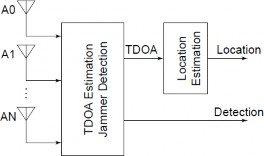Figure 3.2 Two Step processing or Multiplier -correlator TDOA (RD) Estimator

Although this form of localization has intuitive appeal, but is very costly to implement, requiring a search over a nonconvex, difficult-to-compute functional.

3. ### Time difference of arrival (TDOA)

In the case of Range Difference (RD) or TDOA, estimating the vector of inter sensor RDs, often referred to (given scaling by the speed of light or sound) as time differences of arrival (TDOA),

computationally inexpensive Maximum Likelihood (ML) methods are available In this category many studies proposed for example the multisource case, proposed an RD vector estimator implemented as a set of filtered multiplier-correlators, followed by a linear post-processor. This structure is inexpensive to implement and, to first order in the square of the typical sensor noise to signal ratio, is unbiased with variance equal to the Cramer-Rao lower bound (CRB). It should be pointed out that for sensor signal to noise ratios below one, the CRB becomes unachievable. The estimation of position from RDs has been fairly well studied in the passive localization literature for the special case of the source far from an array with collinear elements. Regarding  Hahn derives the Cramer-Rao bound (CRB) and a closed-form maximum likelihood (ML) estimator for the source position given a set of unbiased, CRB-variance RDs. These techniques are made possible by the fact that, under the source-far-from-a-collinear-element-array assumption, the intermediate variables are linear in the measurements. In general, intermediate variables linear in the measurements cannot be found, and the ML estimate will be given as the solution of a difficult nonlinear least-squares problem.

4. ### Generalized interference detection and localization (GIDL)

The last method we introduce here is GIDL detection and localization , Let us assume that we have a system containing two sensors (or antenna) and some signal source (jammer) located far enough away such that it is possible to assume that signal wave fronts from this source are planar with respect to the sensors (antenna) baseline. If differential signal propagation delay time is measured between these two antenna, and signal propagation speed is known in the media (for example, the speed of light), the range difference (RD) measurement (or time difference of arrival (TDOA) measurement, which differs from RD measurements by a scale factor of propagation speed in the media)can be formed. The RDs are equal to the distance between antenna (the baseline) multiplied by the sine of the angle between antenna baseline and direction of the signal wavefronts (direction to the signal source, or direction of propagation, is orthogonal to the direction of wavefronts, and equal to 90 – 𝜃 degrees)

Lsin(𝜃) = 𝜏𝑐 (3.1)

Where c is propagation speed in the media, L is distance between antenna and baseline, 𝜏 is the time difference of arrival (or 𝜏𝑐 is range difference), and 𝜃 is the direction of the wavefront with respect to the baseline. L and c are assumed to be known, and 𝜏 is the measurement. By inverting this equation, it is possible to find direction of the wavefronts 𝜃, or direction to the source 90 −

𝜃.There is an ambiguity in the solution as signal source can be on either side of the baseline, in a

planar case, or on the cone in the case of 3D space. Multiple baselines can resolve this ambiguity. When the assumption concerning a distant source is not valid it is necessary to take into account the fact that wavefronts from this source are spherical. In this case, it is also possible to form the TDOA (or RD) measurement, but now this measurement would define a hyperbola of possible source locations in 2D, or hyperboloid in 3D with antenna in the foci of these hyperbolas. This is shown in Figure 3.3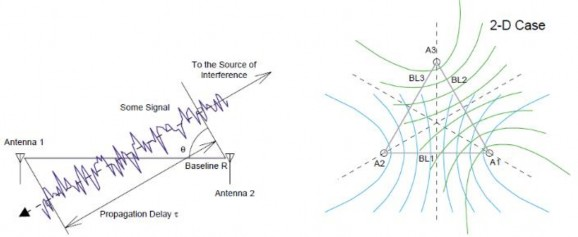Figure 3.3 GIDL Concept: Interferometry and Hyperbolic Localization 

By intersecting hyperbolas from the multiple baselines, it is again possible to find the signal source location in 2D or 3D space. From this simple concept it is easy to see that it is possible to locate the source of interference by means of a completely static system (without moving parts), through the use of omnidirectional antenna plus signal processing algorithms which let us estimate TDOA measurements. However this method had promising results but still there are discussions over accuracy of localization.

2. ### Kalman filter

The Kalman Filter (KF) is a recursive predictive filter that uses a series of measurements observed over time, containing statistical noise and other inaccuracies, and produces estimates of unknown variables that tend to be more precise than those based on a single measurement alone. It estimates the state of a dynamic system. This dynamic system can suffer of some noise mostly white noise. To improve the state predicted according to model, The Kalman filter relies on measurements that are in turn taken into account with an observation model.

Generally speaking Kalman filter consist of two step:

1. The prediction

2. The correction

In first step the state is predicted with dynamic model and in the second step it is corrected with the observation model. So the error covariance of the estimator is minimized. The basic components of Kalman filter are the state vector, Dynamic model and observation model.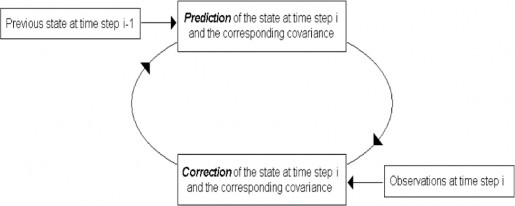Figure 3.4 Kalman filter general circle 

1. ### Least-squares estimation

Discussion around Kalman filter is directed to least-squares estimation theory , from its inception by Gaus’s to its modern form, as developed by Kalman to aid in furnishing the desired perspective, the contributions and insights provided by Gauss are explained and caused developments that have appeared more recently.

Gauss suggested that the most appropriate values for the unknown but desired parameters are the most probable values which he defined in the following manner:” most probable value of the unknown quantities will be that in which the sum of the squares of the differences be that in which the sum of the squares of the differences between the actually observed and the computed values multiplied by numbers that measure the degree of precision is a minimum” The difference between the observed and computed measurement values is generally called the Residual. To show this statement more precise suppose that m measurement quantities are available at discrete instants of time (𝑡1, 𝑡2, … , 𝑡𝑛) and are denoted at each time 𝑡𝑘 as 𝑧𝑘. Suppose we want to determine parameters x from data which they are related according to:

𝑧𝑘 = 𝐻𝑘x + 𝑣𝑘 (3.2)

where 𝑣𝑘 represent the measurement errors that occur at each observation time and 𝐻𝑘 is observation matrix. As we can see from equation the measurement data and the parameters x are assumed here to be linearly related, therefore making explicit the assumption that Gauss indicated was necessary in the foregoing quotation. Denote the estimate of x based on the n data samples

{𝑧1, 𝑧2, … , 𝑧𝑛} as 𝑥̂𝑛 . Then the residual associated with kth measurement is:

𝑟𝑘 = 𝑧𝑘 − 𝐻𝑘𝑥̂𝑛 k = 0, 1, … , n (3.3)

The least squares method is concerned with determining the most probable value of x. This most probable value is defined as the value that minimizes the sum of the squares of the residuals. Thus choose x so that following equation is minimized:

𝑛

1

𝐿 = ∑[𝑧

− 𝐻

𝒙]𝑇 𝑊 [ 𝑧

− 𝐻

𝒙]

𝑛 2

𝑘 𝑘

𝑘=0

𝑘 𝑘 𝑘

(3.4)

The elements of the matrices 𝑊𝑘 are selected to indicate the degree of confidence that one can place in the individual measurements. As we will explain more in the next discussion 𝑊𝑘 is equivalent to the inverse of the covariance of the measurement noise.

2. ### Kalman filter theory

After discussing Gauss basic theory of estimation now we enter the Kalman filter theory, some of the major developments of estimation theory that preceded the introduction of the Kalman filter, R.A.Fisher introduced the idea of maximum likelihood estimation and this has provided food for thought throughout the subsequent years . The system we considering is composed of two essential ingredients. First the state is assumed to be described by:

𝒙𝑘+1 = 𝐴𝑘+1,𝑘𝒙𝑘 + 𝒘𝑘 (3.5)

where 𝒙𝑘+1 is system state vector at k+1,small 𝒘𝑘 represented a white noise sequence, 𝐴𝑘+1,𝑘 is state transition matrix.

The measurement data that are related to the state yielded by:

𝑦𝑘 = 𝐻𝑘𝒙𝑘 + 𝒗𝑘 (3.6)

Where 𝒗𝑘 represent independent white-noise sequences. The initial state 𝒙0 has a mean value

𝑥̂0/−1 and covariance matrix 𝑃̂0/−1. The noise sequences have zero mean and second-order statistics described by:

𝐸[𝑣𝑘𝑣𝑗𝑇] = 𝑅𝑘𝛿𝑘𝑗

𝐸[𝑤𝑘𝑤𝑗𝑇] = 𝑄𝑘𝛿𝑘𝑗

(3.7)

(3.8)

𝐸[𝑣𝑘

𝑤𝑗𝑇] = 0 for all k , j

(3.9)

Where 𝛿𝑘𝑗 is the Kronecker Delta. An estimate 𝑥̂𝑘/𝑘 of the state 𝑥𝑘 is to be computed from the data 𝑦1, 𝑦2, … , 𝑦𝑛 so as to minimize the mean-square error in the estimate. The estimate that accomplishes this is to be computed as an explicit function only of the measurement 𝑦𝑘 and the previous best estimate 𝑥̂𝑘−1/ 𝑘−1.This approach leads to a recursive solution that provides an estimate that is equivalent to the estimate obtained by processing all of the data simultaneously but reduces the data-handling requirements.

The estimate of the signal

𝑠𝑘 = 𝐻𝑘𝑥𝑘 (3.10)

Is given by:

𝑠̂𝑘/𝑘 = 𝐻𝑘𝑥̂𝑘/𝑘 (3.11)

The solution of this recursive linear mean square estimation problem can be determined from the orthogonality principle or any other ways and presented below. This system of equations has come

to be known as the Kalman filter. The estimate is given as the linear combination of the estimate predicted in the absence of new data and the residual 𝑟𝑘 .

𝑥̂𝑘/𝑘−1 = 𝐴𝑘,𝑘−1𝑥̂𝑘−1/𝑘−1 (3.12)

Thus the mean square estimate is:

𝑥̂𝑘/𝑘 = 𝐴𝑘,𝑘−1 𝑥̂𝑘−1/𝑘−1 + 𝐾𝑘[𝑦𝑘 − 𝐻𝑘𝐴𝑘,𝑘−1𝑥̂𝑘−1/𝑘−1 ] (3.13)

The gain matrix 𝐾𝑘 can be considered as being chosen to minimize 𝐸[(𝑥̂𝑘 − 𝑥̂𝑘/𝑘 )𝑇(𝑥𝑘 − 𝑥𝑘/𝑘)] and is given by:

𝐾 = 𝑃 𝐻𝑇(𝐻 𝑃 𝐻𝑇 + 𝑅

)−1 (3.14)

𝑘 𝑘/𝑘−1 𝑘 𝑘 𝑘/𝑘−1 𝑘 𝑘

The matrix 𝑃𝑘/𝑘−1 is the covariance of the error in the predicted estimate and is given by:

𝑃𝑘/𝑘−1 = 𝐸[(𝑥̂𝑘 − 𝑘/𝑘 − 1)𝑇(𝑥𝑘 − 𝑥𝑘/𝑘−1) (3.15)

= 𝐴𝑘/𝑘−1𝑃𝑘−1/𝑘−1𝐴𝑘/𝑘−1𝑇 + 𝑄𝑘−1 (3.16) The 𝑃𝑘/𝑘 is the covariance of the error in the estimate 𝑥̂𝑘/𝑘 .

𝑃𝑘/𝑘

= 𝐸[(𝑥̂𝑘

− 𝑥̂

𝑇

𝑘/𝑘)

(𝑥𝑘 − 𝑥𝑘/𝑘

)] (3.17)

= 𝑃𝑘/𝑘−1 − 𝐾𝑘𝐻𝑘𝑃𝑘/𝑘−1 (3.18)

First Kalman assumes that the noise is independent from one sampling time to the next one, this is equivalent to say that the residual 𝑦𝑘 − 𝑥𝑘𝐻𝑘 is independent between sampling times and therefore agrees with Gauss assumption. Kalman assumed the noise and initial state are essentially Gaussian. The linearity of the system causes the state and measurements to be Gaussian at each sampling time. Thus the residual is Guassian too. So the basic assumption of Gauss and Kalman

is the same except that latter allows the state to change from one time to the next. To reduce approximate errors, the so called “extended Kalman filter” is generally used in practice. In this case the nonlinear system is linearized by employing the best estimates of the state vector as the reference values used at each stage for the linearization. As an example at the time 𝑡𝑘−1, the estimate 𝑥̂𝑘−1/𝑘−1 is used as the reference in obtaining the transition matrix 𝐴𝑘/𝑘−1 . This

approximation is utilized in 𝑃𝑘/𝑘−1 = 𝐴𝑘/𝑘−1 𝑃𝑘−1/𝑘−1 𝐴𝑘/𝑘−1𝑇 + 𝑄𝑘−1 to obtain Kalman error covariance 𝑃𝑘/𝑘−1. The estimate is given by:

𝑥̂𝑘/𝑘−1 = 𝑓𝑘(𝑥̂𝑘−1/𝑘−1) (3.19)

Where 𝑓𝑘 is used to denote the nonlinear plant equation. The estimate 𝑥̂𝑘/𝑘−1 serves as the reference for the determination of a linear approximation 𝐻𝑘 to the nonlinear measurement equation. The filtered estimate is then given by:

𝑥̂𝑘/𝑘 = 𝑥̂𝑘/𝑘−1 + 𝐾𝑘[𝑧𝑘 − ℎ𝑘(𝑥̂𝑘/𝑘−1)] (3.20)

Where 𝑘 is used to denote the measurement nonlinearity. That measurement is assumed to be described by:

𝑦𝑘 = ℎ𝑘(𝑥𝑘) + 𝑣𝑘 (3.21)

The Extended Kalman Filter (EKF) is the nonlinear version of the Kalman filter which linearizes about an estimate of the current mean and covariance. Through the use of the extended Kalman filter one can hope to eliminate or reduce divergence. Note, however, that the 𝑃𝑘/𝑘−1 𝑎𝑛𝑑 𝑃𝑘/𝑘 matrices are still linear approximations of the true error covariance matrices. Further, the nonlinear model 𝑓𝑘and 𝑘 are themselves approximations of the actual physical system so modeling error can still exist thus the extended Kalman filter does not insure the elimination of the divergence problem.

3. ### Algorithm description

The proposed algorithm may be applied to different contexts, whenever detection and estimation problems must be solved simultaneously. Joint jammer detection and localization  algorithm consists of three main steps:

1. Energy detection

2. Aggregate decision

3. Localization

1. ### Energy detection

First step dedicated to measuring received power and take a partial decision on the absence or presence of an interfering signal.

In this algorithm we will use energy detection method which described in previous chapters (2.2.2), however other detection methods could also deployed. Here we make a connection between described method in 2.2.2 and proposed algorithm. Therefore, We can consider received signal vector as 𝑟𝑖:

𝑟𝑖

= {𝑛𝑖 𝑖𝑓 𝐻0(𝑛𝑜 𝑖𝑛𝑡𝑒𝑟𝑓𝑒𝑟𝑒𝑛𝑐𝑒)

𝑠𝑟,𝑖 𝑖𝑓 𝐻1( 𝑖𝑛𝑡𝑒𝑟𝑓𝑒𝑟𝑒𝑛𝑐𝑒 )

(3.22)

Where 𝑛𝑖 is noise samples which they are independent and identically distributed. Each node equipped with an Energy Detector (ED) and has this capability of individually detecting whether the interferer is present (𝐻̂1) or absent 𝐻̂0. The probability of false alarm (𝑃𝑓𝑎) is constant and observable 𝑦𝑖 use received power according to partial decision as:

𝑦𝑖 =

𝑐 4𝜋𝑓𝑐

𝛼

(

𝑇 𝑟𝑖

𝑃𝑡

) − 𝑃𝑁

𝑖𝑓𝐻̂1

{𝑌 𝑖𝑓 𝐻̂0 (3.23)

Where 𝑇(𝑟𝑖) is defined in equation (2.7), 𝑃𝑡 is transmitting power, 𝑃𝑁 is noise power, 𝑓𝑐 is carrier frequency. For this experiment we set 𝛼 = 2 by assuming the free-space path loss model. The interferer transmit power in equation above is unknown therefore it is not possible to provide accurate distance estimations between nodes and jammer at detection stage. Hence, we assumes a transmit power of 1W. This simplifying assumption leads to inevitable errors in the interferer's position estimation, which however do not affect detection performance. This issue of positioning

accuracy is later resumed and addressed by the localization stage of the algorithm. The energy detection operation is depicted in Figure 3.5.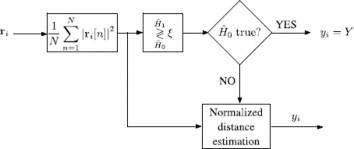Figure 3.5 Energy detector 

2. ### Aggregate decision

Now we move to second step mentioned as aggregate decision, The detection Kalman filter's unknown state vector to be estimated is composed by the jammer's coordinates 𝐱 = (X, Y)𝑇 .We suppose a stationary transition model with an identity matrix 𝑨 = 𝐈𝟐 for each time instant, which is equivalent of assuming a fixed interferer location. Furthermore, no controlled input signals are considered in the system 𝐁 = 𝟎. As far as observations are concerned, the nonlinear relation connecting measurements and the state vector is given by:

𝑖

𝐷(𝑥) = √(𝑋𝑖 − 𝑋 )2 + (𝑌𝑖 − 𝑌 )2 (3.24)

where (𝑋𝑖, 𝑌𝑖) is nodes position in Cartesian coordinate, 𝐇𝐷 is observation model of size 2 × 𝑀

𝑖

is described by the Jacobian matrix of 𝐷:

𝑋1 − 𝑋

l √(𝑋1 − 𝑋)2 + (𝑌1 − 𝑌)2

𝑌1 − 𝑌

√(𝑋1 − 𝑋)2 + (𝑌1 − 𝑌)2

𝐇𝐷 =

⋮ ⋮

𝑋𝑀 − 𝑋

𝗁 √(𝑋𝑀 − 𝑋)2 + (𝑌𝑀 − 𝑌)2

𝑌𝑀 − 𝑌

√(𝑋𝑀 − 𝑋)2 + (𝑌𝑀 − 𝑌)2)